# JP6024129B2 - Non-contact power feeding device - Google Patents

## Info

Publication number
JP6024129B2
JP6024129B2 JP2012055749A JP2012055749A JP6024129B2 JP 6024129 B2 JP6024129 B2 JP 6024129B2 JP 2012055749 A JP2012055749 A JP 2012055749A JP 2012055749 A JP2012055749 A JP 2012055749A JP 6024129 B2 JP6024129 B2 JP 6024129B2
Authority
JP
Japan
Prior art keywords
power
command value
output
unit
means
Prior art date
Legal status (The legal status is an assumption and is not a legal conclusion. Google has not performed a legal analysis and makes no representation as to the accuracy of the status listed.)
Active
Application number
JP2012055749A
Other languages
Japanese (ja)
Other versions
JP2013192326A (en
Inventor

クライソン　トロンナムチャイ
トロンナムチャイ クライソン

Original Assignee

Priority date (The priority date is an assumption and is not a legal conclusion. Google has not performed a legal analysis and makes no representation as to the accuracy of the date listed.)
Filing date
Publication date
Application filed by 日産自動車株式会社 filed Critical 日産自動車株式会社
Priority to JP2012055749A priority Critical patent/JP6024129B2/en
Publication of JP2013192326A publication Critical patent/JP2013192326A/en
Application granted granted Critical
Publication of JP6024129B2 publication Critical patent/JP6024129B2/en
Application status is Active legal-status Critical
Anticipated expiration legal-status Critical

## Images

•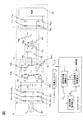•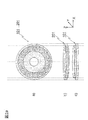•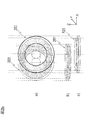•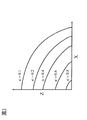•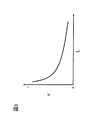•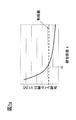••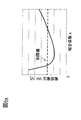•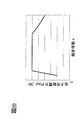•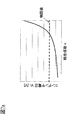•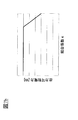•••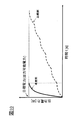•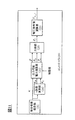•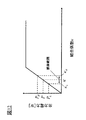•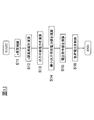•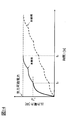•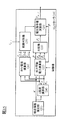•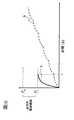•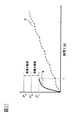•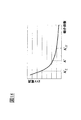•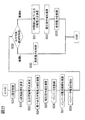•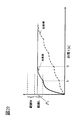•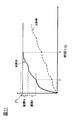•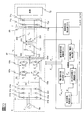•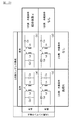•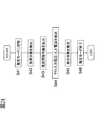•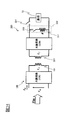•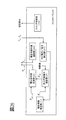•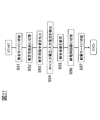•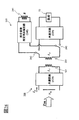•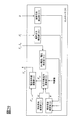•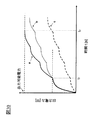•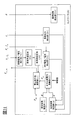•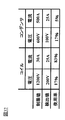•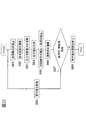•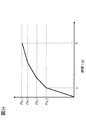•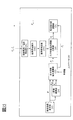•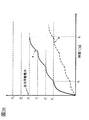•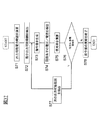## Classifications

• BPERFORMING OPERATIONS; TRANSPORTING
• B60VEHICLES IN GENERAL
• B60LPROPULSION OF ELECTRICALLY-PROPELLED VEHICLES; SUPPLYING ELECTRIC POWER FOR AUXILIARY EQUIPMENT OF ELECTRICALLY-PROPELLED VEHICLES; ELECTRODYNAMIC BRAKE SYSTEMS FOR VEHICLES IN GENERAL; MAGNETIC SUSPENSION OR LEVITATION FOR VEHICLES; MONITORING OPERATING VARIABLES OF ELECTRICALLY-PROPELLED VEHICLES; ELECTRIC SAFETY DEVICES FOR ELECTRICALLY-PROPELLED VEHICLES
• B60L53/00Methods of charging batteries, specially adapted for electric vehicles; Charging stations or on-board charging equipment therefor; Exchange of energy storage elements in electric vehicles
• B60L53/10Methods of charging batteries, specially adapted for electric vehicles; Charging stations or on-board charging equipment therefor; Exchange of energy storage elements in electric vehicles characterised by the energy transfer between the charging station and the vehicle
• B60L53/12Inductive energy transfer
• BPERFORMING OPERATIONS; TRANSPORTING
• B60VEHICLES IN GENERAL
• B60LPROPULSION OF ELECTRICALLY-PROPELLED VEHICLES; SUPPLYING ELECTRIC POWER FOR AUXILIARY EQUIPMENT OF ELECTRICALLY-PROPELLED VEHICLES; ELECTRODYNAMIC BRAKE SYSTEMS FOR VEHICLES IN GENERAL; MAGNETIC SUSPENSION OR LEVITATION FOR VEHICLES; MONITORING OPERATING VARIABLES OF ELECTRICALLY-PROPELLED VEHICLES; ELECTRIC SAFETY DEVICES FOR ELECTRICALLY-PROPELLED VEHICLES
• B60L53/00Methods of charging batteries, specially adapted for electric vehicles; Charging stations or on-board charging equipment therefor; Exchange of energy storage elements in electric vehicles
• B60L53/10Methods of charging batteries, specially adapted for electric vehicles; Charging stations or on-board charging equipment therefor; Exchange of energy storage elements in electric vehicles characterised by the energy transfer between the charging station and the vehicle
• B60L53/12Inductive energy transfer
• B60L53/126Methods for pairing a vehicle and a charging station, e.g. establishing a one-to-one relation between a wireless power transmitter and a wireless power receiver
• HELECTRICITY
• H02GENERATION; CONVERSION OR DISTRIBUTION OF ELECTRIC POWER
• H02JCIRCUIT ARRANGEMENTS OR SYSTEMS FOR SUPPLYING OR DISTRIBUTING ELECTRIC POWER; SYSTEMS FOR STORING ELECTRIC ENERGY
• H02J5/00Circuit arrangements for transfer of electric power between ac networks and dc networks
• H02J5/005Circuit arrangements for transfer of electric power between ac networks and dc networks with inductive power transfer
• HELECTRICITY
• H02GENERATION; CONVERSION OR DISTRIBUTION OF ELECTRIC POWER
• H02JCIRCUIT ARRANGEMENTS OR SYSTEMS FOR SUPPLYING OR DISTRIBUTING ELECTRIC POWER; SYSTEMS FOR STORING ELECTRIC ENERGY
• H02J50/00Circuit arrangements or systems for wireless supply or distribution of electric power
• H02J50/10Circuit arrangements or systems for wireless supply or distribution of electric power using inductive coupling
• H02J50/12Circuit arrangements or systems for wireless supply or distribution of electric power using inductive coupling of the resonant type
• HELECTRICITY
• H02GENERATION; CONVERSION OR DISTRIBUTION OF ELECTRIC POWER
• H02JCIRCUIT ARRANGEMENTS OR SYSTEMS FOR SUPPLYING OR DISTRIBUTING ELECTRIC POWER; SYSTEMS FOR STORING ELECTRIC ENERGY
• H02J50/00Circuit arrangements or systems for wireless supply or distribution of electric power
• H02J50/90Circuit arrangements or systems for wireless supply or distribution of electric power involving detection or optimisation of position, e.g. alignment
• HELECTRICITY
• H02GENERATION; CONVERSION OR DISTRIBUTION OF ELECTRIC POWER
• H02JCIRCUIT ARRANGEMENTS OR SYSTEMS FOR SUPPLYING OR DISTRIBUTING ELECTRIC POWER; SYSTEMS FOR STORING ELECTRIC ENERGY
• H02J7/00Circuit arrangements for charging or depolarising batteries or for supplying loads from batteries
• H02J7/02Circuit arrangements for charging or depolarising batteries or for supplying loads from batteries for charging batteries from ac mains by converters
• H02J7/022Circuit arrangements for charging or depolarising batteries or for supplying loads from batteries for charging batteries from ac mains by converters characterised by the type of converter
• H02J7/025Circuit arrangements for charging or depolarising batteries or for supplying loads from batteries for charging batteries from ac mains by converters characterised by the type of converter using non-contact coupling, e.g. inductive, capacitive
• BPERFORMING OPERATIONS; TRANSPORTING
• B60VEHICLES IN GENERAL
• B60LPROPULSION OF ELECTRICALLY-PROPELLED VEHICLES; SUPPLYING ELECTRIC POWER FOR AUXILIARY EQUIPMENT OF ELECTRICALLY-PROPELLED VEHICLES; ELECTRODYNAMIC BRAKE SYSTEMS FOR VEHICLES IN GENERAL; MAGNETIC SUSPENSION OR LEVITATION FOR VEHICLES; MONITORING OPERATING VARIABLES OF ELECTRICALLY-PROPELLED VEHICLES; ELECTRIC SAFETY DEVICES FOR ELECTRICALLY-PROPELLED VEHICLES
• B60L53/00Methods of charging batteries, specially adapted for electric vehicles; Charging stations or on-board charging equipment therefor; Exchange of energy storage elements in electric vehicles
• B60L53/30Constructional details of charging stations
• B60L53/35Means for automatic or assisted adjustment of the relative position of charging devices and vehicles
• B60L53/38Means for automatic or assisted adjustment of the relative position of charging devices and vehicles specially adapted for charging by inductive energy transfer
• B60L53/39Means for automatic or assisted adjustment of the relative position of charging devices and vehicles specially adapted for charging by inductive energy transfer with position-responsive activation of primary coils
• HELECTRICITY
• H02GENERATION; CONVERSION OR DISTRIBUTION OF ELECTRIC POWER
• H02JCIRCUIT ARRANGEMENTS OR SYSTEMS FOR SUPPLYING OR DISTRIBUTING ELECTRIC POWER; SYSTEMS FOR STORING ELECTRIC ENERGY
• H02J2310/00The network for supplying or distributing electric power characterised by its spatial reach or by the load
• H02J2310/40The network being an on-board power network, i.e. within a vehicle
• H02J2310/48The network being an on-board power network, i.e. within a vehicle for electric vehicles [EV] or hybrid vehicles [HEV]
• YGENERAL TAGGING OF NEW TECHNOLOGICAL DEVELOPMENTS; GENERAL TAGGING OF CROSS-SECTIONAL TECHNOLOGIES SPANNING OVER SEVERAL SECTIONS OF THE IPC; TECHNICAL SUBJECTS COVERED BY FORMER USPC CROSS-REFERENCE ART COLLECTIONS [XRACs] AND DIGESTS
• Y02TECHNOLOGIES OR APPLICATIONS FOR MITIGATION OR ADAPTATION AGAINST CLIMATE CHANGE
• Y02TCLIMATE CHANGE MITIGATION TECHNOLOGIES RELATED TO TRANSPORTATION
• Y02T10/00Road transport of goods or passengers
• Y02T10/60Other road transportation technologies with climate change mitigation effect
• Y02T10/70Energy storage for electromobility
• Y02T10/7005Batteries
• YGENERAL TAGGING OF NEW TECHNOLOGICAL DEVELOPMENTS; GENERAL TAGGING OF CROSS-SECTIONAL TECHNOLOGIES SPANNING OVER SEVERAL SECTIONS OF THE IPC; TECHNICAL SUBJECTS COVERED BY FORMER USPC CROSS-REFERENCE ART COLLECTIONS [XRACs] AND DIGESTS
• Y02TECHNOLOGIES OR APPLICATIONS FOR MITIGATION OR ADAPTATION AGAINST CLIMATE CHANGE
• Y02TCLIMATE CHANGE MITIGATION TECHNOLOGIES RELATED TO TRANSPORTATION
• Y02T10/00Road transport of goods or passengers
• Y02T10/60Other road transportation technologies with climate change mitigation effect
• Y02T10/70Energy storage for electromobility
• Y02T10/7072Electromobility specific charging systems or methods for batteries, ultracapacitors, supercapacitors or double-layer capacitors
• YGENERAL TAGGING OF NEW TECHNOLOGICAL DEVELOPMENTS; GENERAL TAGGING OF CROSS-SECTIONAL TECHNOLOGIES SPANNING OVER SEVERAL SECTIONS OF THE IPC; TECHNICAL SUBJECTS COVERED BY FORMER USPC CROSS-REFERENCE ART COLLECTIONS [XRACs] AND DIGESTS
• Y02TECHNOLOGIES OR APPLICATIONS FOR MITIGATION OR ADAPTATION AGAINST CLIMATE CHANGE
• Y02TCLIMATE CHANGE MITIGATION TECHNOLOGIES RELATED TO TRANSPORTATION
• Y02T90/00Enabling technologies or technologies with a potential or indirect contribution to GHG emissions mitigation
• Y02T90/10Technologies related to electric vehicle charging
• Y02T90/12Electric charging stations
• YGENERAL TAGGING OF NEW TECHNOLOGICAL DEVELOPMENTS; GENERAL TAGGING OF CROSS-SECTIONAL TECHNOLOGIES SPANNING OVER SEVERAL SECTIONS OF THE IPC; TECHNICAL SUBJECTS COVERED BY FORMER USPC CROSS-REFERENCE ART COLLECTIONS [XRACs] AND DIGESTS
• Y02TECHNOLOGIES OR APPLICATIONS FOR MITIGATION OR ADAPTATION AGAINST CLIMATE CHANGE
• Y02TCLIMATE CHANGE MITIGATION TECHNOLOGIES RELATED TO TRANSPORTATION
• Y02T90/00Enabling technologies or technologies with a potential or indirect contribution to GHG emissions mitigation
• Y02T90/10Technologies related to electric vehicle charging
• Y02T90/12Electric charging stations
• Y02T90/121Electric charging stations by conductive energy transmission
• YGENERAL TAGGING OF NEW TECHNOLOGICAL DEVELOPMENTS; GENERAL TAGGING OF CROSS-SECTIONAL TECHNOLOGIES SPANNING OVER SEVERAL SECTIONS OF THE IPC; TECHNICAL SUBJECTS COVERED BY FORMER USPC CROSS-REFERENCE ART COLLECTIONS [XRACs] AND DIGESTS
• Y02TECHNOLOGIES OR APPLICATIONS FOR MITIGATION OR ADAPTATION AGAINST CLIMATE CHANGE
• Y02TCLIMATE CHANGE MITIGATION TECHNOLOGIES RELATED TO TRANSPORTATION
• Y02T90/00Enabling technologies or technologies with a potential or indirect contribution to GHG emissions mitigation
• Y02T90/10Technologies related to electric vehicle charging
• Y02T90/12Electric charging stations
• Y02T90/122Electric charging stations by inductive energy transmission
• YGENERAL TAGGING OF NEW TECHNOLOGICAL DEVELOPMENTS; GENERAL TAGGING OF CROSS-SECTIONAL TECHNOLOGIES SPANNING OVER SEVERAL SECTIONS OF THE IPC; TECHNICAL SUBJECTS COVERED BY FORMER USPC CROSS-REFERENCE ART COLLECTIONS [XRACs] AND DIGESTS
• Y02TECHNOLOGIES OR APPLICATIONS FOR MITIGATION OR ADAPTATION AGAINST CLIMATE CHANGE
• Y02TCLIMATE CHANGE MITIGATION TECHNOLOGIES RELATED TO TRANSPORTATION
• Y02T90/00Enabling technologies or technologies with a potential or indirect contribution to GHG emissions mitigation
• Y02T90/10Technologies related to electric vehicle charging
• Y02T90/12Electric charging stations
• Y02T90/125Alignment between the vehicle and the charging station
• YGENERAL TAGGING OF NEW TECHNOLOGICAL DEVELOPMENTS; GENERAL TAGGING OF CROSS-SECTIONAL TECHNOLOGIES SPANNING OVER SEVERAL SECTIONS OF THE IPC; TECHNICAL SUBJECTS COVERED BY FORMER USPC CROSS-REFERENCE ART COLLECTIONS [XRACs] AND DIGESTS
• Y02TECHNOLOGIES OR APPLICATIONS FOR MITIGATION OR ADAPTATION AGAINST CLIMATE CHANGE
• Y02TCLIMATE CHANGE MITIGATION TECHNOLOGIES RELATED TO TRANSPORTATION
• Y02T90/00Enabling technologies or technologies with a potential or indirect contribution to GHG emissions mitigation
• Y02T90/10Technologies related to electric vehicle charging
• Y02T90/14Plug-in electric vehicles

## Description

The present invention relates to a non-contact power feeding device.

Prior to supplying power to the load device, the load device is electrically disconnected, passive elements are connected in parallel, and the output voltage of the variable voltage high frequency power supply is set to the low voltage Vm for measurement. The output current value Im is measured. Using the Vm and Im, the voltage VIN of the variable voltage high frequency power source for setting the output voltage of the secondary coil or rectifier to the target value when the load device is connected to the secondary coil is calculated. Even if the communication device does not exchange information between the primary side and the secondary side, the voltage of the variable voltage high frequency power supply 1 is controlled to set the voltage of the secondary coil or the output voltage of the rectifier to the target value. A non-contact power feeding device is known (Patent Document 1).

JP 2011-45195 A

However, in the above non-contact power feeding device, the output voltage is set without considering the withstand voltage or the withstand current of the secondary coil, capacitor, etc., so output from the secondary coil based on the withstand voltage is possible. When setting the output power to the power, there is a problem that it takes time to control the voltage, and the time until the output possible power is reached becomes long.

The present invention provides a non-contact power feeding device that can shorten the control time required to reach output power to a load electrically connected to a power receiving coil.

The present invention estimates the coupling state between the power transmission coil and the power reception coil, and can output to the load based on the limit value of the circuit element of the power feeding circuit having the power transmission coil and the power reception coil and the coupling state The above problem is solved by calculating electric power.

According to the present invention, the output power that can be output to the load in consideration of the limit value of the circuit element is grasped by calculation according to the estimated coupling state, so that the control time until the output power is reached is shortened. be able to.

It is an electric circuit diagram of the non-contact electric power feeder of this example. It is the top view and perspective view of the primary winding and secondary winding of FIG. It is the top view and perspective view of the primary winding and secondary winding of FIG. It is a graph which shows the characteristic of the coupling coefficient with respect to the distance of the primary winding and secondary winding of FIG. It is a figure which shows the change of the coupling coefficient with respect to the shift | offset | difference of the secondary winding of FIG. 1 in the height direction. It is a graph which shows the voltage characteristic of the power transmission coil (primary winding) (V1) with respect to the coupling coefficient ((kappa)) in the non-contact electric power feeding part of FIG. Is a graph showing power characteristics of the available output power (P out) for the coupling coefficient (kappa) in a non-contact power supply unit of FIG. In the non-contact power supply unit according to a modification of the present invention, it is a graph showing voltage characteristics of the input voltage to the primary side of the non-contact power feeding section 10 to the coupling coefficient (κ) (V in). In the non-contact power supply unit according to a modification of the present invention, it is a graph illustrating the power characteristic of the available output power (P out) for the coupling coefficient (kappa). In the non-contact power supply unit according to a modification of the present invention, it is a graph showing a characteristic of the voltage of the capacitor 202 (V c) with respect to the coupling coefficient (kappa). In the non-contact power supply unit according to a modification of the present invention, it is a graph illustrating the power characteristic of the available output power (P out) for the coupling coefficient (kappa). In a comparative example, it is a graph which shows the time characteristic of the output electric power to a load part. It is a flowchart which shows the control procedure of the controller of FIG. The time characteristic of the output electric power in the non-contact electric power feeder which concerns on this embodiment and a comparative example is shown. It is a block diagram of the controller of the non-contact electric power supply which concerns on other embodiment of this invention. It is a figure explaining the error range of the coupling coefficient of the non-contact electric power feeding controlled by the controller of FIG. It is a flowchart which shows the control procedure of the controller of FIG. The time characteristic of the output electric power in the non-contact electric power feeder which concerns on this embodiment and a comparative example is shown. It is a block diagram of the controller of the non-contact electric power supply which concerns on other embodiment of this invention. It is a graph which shows the output electric power characteristic to the load part 7 with respect to time in the non-contact electric power supply controlled by the controller of FIG. It is a graph which shows the output electric power characteristic to the load part 7 with respect to time in the non-contact electric power supply controlled by the controller of FIG. 16 is a graph showing the characteristics of the inverter current with respect to the coupling coefficient in the non-contact power feeding apparatus controlled by the controller of FIG. It is a flowchart which shows the control procedure of the controller of FIG. The time characteristic of the output electric power in the non-contact electric power feeder which concerns on this embodiment and a comparative example is shown. The time characteristic of the output electric power in the non-contact electric power feeder which concerns on this embodiment and a comparative example is shown. It is a block diagram of the non-contact electric power feeder which concerns on other embodiment of this invention. It is a figure for demonstrating the relationship between the typical circuit structure of the non-contact electric power feeding part of FIG. 22, and resonance conditions. It is a flowchart which shows the control procedure of the controller of FIG. It is a circuit diagram of the non-contact electric power feeding part and load of the non-contact electric power feeder which concerns on other embodiment of this invention. It is a block diagram of the controller of the non-contact electric power feeder provided with the circuit of FIG. It is a flowchart which shows the control procedure of the controller of FIG. It is a circuit diagram of the non-contact electric power feeding part and load of the non-contact electric power feeder which concerns on other embodiment of this invention. It is a block diagram of the controller of the non-contact electric power feeder provided with the circuit of FIG. The time characteristic of the output electric power in the non-contact electric power feeder which concerns on this embodiment and a comparative example is shown. It is a block diagram of the controller of the non-contact electric power supply which concerns on other embodiment of this invention. It is a figure which shows the relationship between the applied voltage of the primary winding and capacitor | condenser of FIG. FIG. 32 is a flowchart showing a control procedure of the controller of FIG. 31. FIG. The time characteristic of the output electric power in the non-contact electric power feeder which concerns on this embodiment is shown. It is a block diagram of the controller of the non-contact electric power supply which concerns on other embodiment of this invention. The time characteristic of the output electric power in the non-contact electric power feeder which concerns on this embodiment and a comparative example is shown. It is a flowchart which shows the control procedure of the controller of FIG.

Hereinafter, embodiments of the present invention will be described with reference to the drawings.
<< First Embodiment >>
As an example of a non-contact power supply apparatus according to an embodiment of the invention, a non-contact power supply apparatus used together with a battery for a vehicle such as an electric vehicle and a power load will be described.

FIG. 1 shows an electric circuit diagram of the non-contact power feeding device. The non-contact power feeding device according to the present embodiment is supplied with power by the high-frequency AC power source 6, the non-contact power feeding unit 10 that performs non-contact power feeding of power output from the high-frequency AC power source 6, and the non-contact power feeding unit 10. The load part 7 is provided.

The high-frequency AC power supply 6 is connected to the three-phase AC power supply 64, the three-phase AC power supply 64, the rectifier 61 that rectifies the three-phase AC to DC, and is connected to the rectifier 61 via the smoothing capacitor 62. And a voltage type inverter 63 that reversely converts the power to high frequency power. The rectifier 61 connects the diode 61a and the diode 61b, the diode 61c and the diode 61d, and the diode 61e and the diode 61f in parallel, and connects the output of the three-phase AC power supply 64 to each intermediate connection point. The voltage type inverter 63 connects in parallel a series circuit of a switching element 63b similar to a switching element 63a that connects a diode to a MOSFET power transistor or the like in antiparallel, and a series circuit of a similar switching element 63c and switching element 63d. Then, it is connected to the rectifier 61 through the smoothing capacitor 62. Then, an intermediate connection point between the switching element 63a and the switching element 63b and an intermediate connection point between the switching element 63c and the switching element 63d are connected to the power transmission circuit unit 100 that is the primary side of the non-contact power feeding unit 10, respectively. The voltage type inverter 63 supplies AC power of about several k to 100 kHz to the non-contact power feeding unit 100.

The non-contact power feeding unit 10 includes a power transmission circuit unit 100 that is an input side of a transformer and a power receiving circuit unit 200 that is an output side of the transformer. The power transmission circuit unit 100 includes a primary winding (L 1 ) 101 and a capacitor (C 1s ) 102 connected in series to the primary winding 101, and the power receiving circuit unit 200 includes a secondary winding (L 2 ). 201 and a capacitor (C 2s ) 202 connected in series to the secondary winding 201.

The load unit 7 includes a rectification unit 71 that rectifies AC power supplied from the non-contact power supply unit 10 into a direct current, and a load 72 that is connected to the rectification unit 71. The rectifying unit 71 connects the diode 71a and the diode 71b, and the diode 71c and the diode 71d in parallel, and connects the output of the power receiving circuit unit 200 to each intermediate connection point. Then, the output of the rectifying unit 71 is connected to the load 72. The load 72 is a load such as a battery.

The controller 20 is a control unit that controls the entire non-contact power feeding apparatus of this example, and includes a coupling coefficient estimation unit 21, an outputable power calculation unit 22, and a voltage command value calculation unit 23. The coupling coefficient estimator 21 estimates the coupling coefficient (κ) between the primary winding 101 and the secondary winding 201 from the detection value of the position sensor 99, so that the primary winding 101 and the secondary winding 201 are estimated. Estimate the coupling state between The coupling coefficient estimator 21 transmits the estimated coupling coefficient (κ) to the outputable power calculator 22.

The output possible voltage power calculation unit 22 is an output that can be output to the load unit 7 based on a limit value such as voltage and current of the primary winding 101 included in the contactless power supply unit 10 and a coupling coefficient (κ). The possible power (P out ) is calculated, and the output possible power (P out ) is transmitted to the voltage command value calculation unit 23.

Here, the limit value and output power (P out ) will be described. The limit value is a value determined in advance according to the withstand voltage and withstand current of each element forming the non-contact power feeding unit 10, the withstand voltage of the inverter 63, the power capacity of the AC power supply 64, and the like. For example, the withstand voltages of the primary winding 101 and the secondary winding 201 are values determined by safety regulations for high voltage, and the withstand voltage is a value determined by the coil diameter, thickness, and the like. Further, the withstand voltage of the capacitors 102 and 202 is determined by the withstand voltage of the capacitor, and the withstand voltage or current withstand of the inverter 63 is determined in advance by the element withstand voltage and the element allowable current of the transistors 63a to 63d.

The output possible voltage (P out ) is a non-contact state in which the current or current applied to the circuit element of the non-contact power feeding unit 10 and the circuit element of the inverter 63 is set to the above limit value or less during the driving of the non-contact power feeding device. The maximum power that can be output from the power supply unit 10 to the load unit 7 is shown. In other words, the output possible voltage (P out ) is the non-contact power feeding unit when the voltage or current of at least one of the circuit elements included in the non-contact power feeding unit 10 and the inverter 63 corresponds to the limit value. 10 output power. Note that the case where the voltage or current of one circuit element corresponds to the limit value means that the voltage or current of the circuit element is equal to the limit value, or that the voltage or current of the circuit element has a margin with respect to the limit value. Is included, and is equal to the lower value.

The voltage command value calculator 23 is a voltage command value (V in_ref ) for causing the load power 7 to output the required power (P out_ref ) or the outputable power (P out ) required from the load. Is output to the inverter 63. The voltage command value corresponds to the switching signal of the transistors 63a to 63d.

The position sensor 99 is a sensor that detects a relative position between the primary winding 101 and the secondary winding. For example, the position sensor 99 is provided in the vicinity of the primary winding 101 and uses a radio wave such as infrared rays to transmit the secondary winding. The position of 202 is measured.

Next, the coupling coefficient (κ) between the primary winding 101 and the secondary winding 201 when the non-contact power feeding device shown in FIG. 1 is provided in a vehicle and a parking lot will be described with reference to FIGS.

In this example, the power receiving circuit unit 200 including the secondary winding 201 and the load unit 7 are provided in a vehicle, for example, and the power transmission circuit unit 100 including the primary winding 101 and the high-frequency AC power supply 6 are provided on the ground side, for example, in a parking lot. In the case of an electric vehicle, the load 72 corresponds to, for example, a secondary battery. The secondary winding 201 is provided, for example, in a vehicle chassis. Then, the driver of the vehicle parks in the parking lot so that the secondary winding 201 is above the primary winding 101, and electric power is supplied from the primary winding 101 to the secondary winding 201, and the load 72 The included secondary battery is charged.

2a and 2b show a plan view and a perspective view of the primary winding 101 and the secondary winding 201. FIG. 2a and 2b, the X axis and the Y axis indicate the planar directions of the primary winding 101 and the secondary winding 201, and the Z axis indicates the height direction. 2A and 2B, a) is a plan view of the primary winding 101 and the secondary winding 201, b) is a perspective view of the secondary winding 201, and c) is a perspective view of the primary winding 101. Indicates. For the sake of explanation, the primary winding 101 and the secondary winding 201 have both the same circular shape. However, in this example, the primary winding 101 and the secondary winding 201 are not necessarily circular. Need not have the same shape.

As shown in FIG. 2a, the vehicle may be parked in the parking lot so that the secondary winding 201 matches the primary winding 101 in the X-axis and Y-axis directions which are planar directions. Therefore, as shown in FIG. 2b, the relative positions of the primary winding 101 and the secondary winding 201 may be shifted in the planar direction. Moreover, since the height of a vehicle changes with kinds of vehicles, the height of the primary winding 101 and the secondary winding 201 also changes with vehicle height.

FIG. 3 shows a change in the coupling coefficient for the secondary winding 201 in the X-axis direction (Y-axis direction) and the Z-axis direction shown in FIGS. 2a and 2b. FIG. 4 shows changes in the coupling coefficient κ with respect to the displacement of the secondary winding 201 in the Z-axis direction shown in FIG. Note that the horizontal axis L in FIG. 4 is expressed by Equation (1) using the planar direction (X-axis direction) and the height direction (Z-axis direction) of the secondary winding 202, and is fixed to the ground. This is the distance of the secondary winding 202 to the winding 101.

As shown in FIG. 2a, when the center of the primary winding 1 and the center of the secondary winding 2 coincide, the leakage magnetic flux between the primary winding 101 and the secondary winding 201 is small, and the X axis of FIG. Is equal to zero, and the coupling state κ increases because the coupling state between the primary winding 101 and the secondary winding 201 is high. On the other hand, as shown in FIG. 2b with respect to FIG. 2a, when the positions of the primary winding 1 and the secondary winding 2 are shifted in the X-axis direction, the leakage flux increases, and the primary winding 101 and the secondary winding Since the coupling state with 201 is low, the mutual inductance M or the coupling coefficient κ is small as shown in FIG. Further, when the displacement in the height direction between the primary winding 101 and the secondary winding 201 increases, the mutual inductance M or the coupling coefficient k decreases as shown in FIG.

Next, the voltage of the primary winding 101 and the output power (P out ) with respect to the coupling coefficient (κ) will be described with reference to FIGS. 5a and 5b. FIG. 5a is a graph showing the voltage characteristics of the power transmission coil (primary winding) (V1) with respect to the coupling coefficient (κ), and FIG. 5b is a graph showing the power characteristics of the output power (P out ) with respect to the coupling coefficient (κ). It is. When the power (P c ) supplied to the load unit 7 is constant, when the coupling coefficient (κ) corresponding to the positional relationship of the coils is changed, as shown in FIG. The voltage of winding 101 has dropped. The limit value, which is the withstand voltage of the primary winding 101, is shown in FIG. 5a.

That is, when the coupling coefficient (kappa) is lower than the kappa a, when to output a constant power (Pc) with respect to the load unit 7, the voltage of the primary winding 101 exceeds the limit value, the actual present example In this system, the voltage of the primary winding 101 cannot be raised higher than the limit value, and the output power (P out ) is lower than the constant power (Pc).

Next, when the coupling coefficient (κ) changes under the condition that the voltage and current applied to the circuit elements of the non-contact power supply unit 10 including the primary winding 101 are less than the limit values, the output to the load unit 7 The possible power (P out ) is represented in FIG. Even when the coupling coefficient (κ) is high, the output power (P out ) is limited by the limit value of each circuit element.

Next, as a modification of the contactless power feeding unit 10 according to this example, a coupling coefficient (κ) in a circuit in which a capacitor 102 is connected in parallel to a primary winding 101 and a capacitor 202 is connected in parallel to a secondary winding 201. The characteristics of the power supply voltage and the power that can be output will be described. 6A is a graph showing the voltage characteristic of the input voltage (V in ) from the high-frequency AC power supply 6 to the primary side of the non-contact power feeding unit 10 with respect to the coupling coefficient (κ), and FIG. 6B is an output with respect to the coupling coefficient (κ). It is a graph which shows the electric power characteristic of possible electric power ( Pout ).

As shown in FIG. 6a, since there is a limit value of the high-frequency AC power supply 6 due to the breakdown voltage of the transistors 63a to 63d, the voltage of the high-frequency AC power supply 6 cannot be increased beyond the limit value. Further, as shown in FIG. 6 b, the output possible voltage (P out ) is limited not only by the coupling coefficient (κ) but also by the limit value of the high-frequency AC power supply 6 or the limit value of the circuit elements of the non-contact power feeding unit 10. I can understand.

As another modification of the non-contact power feeding unit 10 according to this example, a coupling coefficient (in a circuit in which a capacitor 102 is connected in series to a primary winding 101 and a capacitor 202 is connected in parallel to a secondary winding 201 ( A description will be given of the voltage characteristic of the capacitor 202 and the characteristic of outputtable power with respect to κ). FIG. 7A is a graph showing the characteristic of the voltage (V c ) of the capacitor 202 with respect to the coupling coefficient (κ), and FIG. 7B is a graph showing the power characteristic of the output power (P out ) with respect to the coupling coefficient (κ).

As shown in FIG. 7a, since the limit value of the capacitor 202 exists, the voltage of the high-frequency AC power supply 6 cannot be increased beyond the limit value. Further, as shown in FIG. 7b, the output possible voltage (P out ) varies depending on the coupling coefficient (κ), but is limited by the limit value of the capacitor 202 or the like.

That is, as shown in FIG. 5 to FIG. 7, the output possible power (P out ) depends on the limit value of the high-frequency AC power supply 6 and the limit value of the non-contact power supply unit 10 while changing according to the coupling coefficient (κ). Be restricted. 5 to 7, the limit values of the high-frequency AC power supply 6, the primary winding 101, and the capacitor 202 are described. However, the limit values of other circuit elements need to be considered in the same manner.

Next, the voltage and current of each circuit element of the non-contact power feeding unit 10 and the output power to the load unit 7 can be calculated from the circuit parameters of the non-contact power feeding unit 10, the drive frequency of the inverter 63, and the coupling coefficient. The point will be described. In the following expression, the drive frequency of the inverter 63 and the resonance frequency of the non-contact power feeding unit 10 are the same (resonance state).

Ratio of voltage (V in : input voltage of the inverter 63) and current (I in : input current of the inverter 63) input from the high frequency AC power supply 6 to the non-contact power supply unit 10 (input viewed from the high frequency AC power supply 6 side) Impedance) is expressed by the following equation (2).
However, ω represents the drive angular frequency of the inverter 63, L 1 represents the inductance of the primary winding 101, L 2 represents the inductance of the secondary winding 202, and R represents the resistance of the load unit 7.

And the electric current ( Iin ) which flows into the primary side of the non-contact electric power feeding part 10 is represented by the following formula | equation (3).

Then, the voltage (V c1 ) of the capacitor 102 and the voltage (V L1 ) of the primary winding 101 are expressed by Expression (4) and Expression (5), respectively.

Further, the current (I 2 ) flowing to the secondary side of the non-contact power feeding unit 10, the voltage (V C2 ) of the capacitor 202, and the voltage (V L2 ) of the secondary winding 201 are expressed by Equations (6) to (8) It is represented by

Furthermore, the voltage (VR) applied to the load unit 7 and the output power (P o ) to the load unit 7 are expressed by Equation (9) and Equation (10).

Since the circuit parameters of the non-contact power feeding unit 10 are determined at the design stage and are known values, if the coupling coefficient (κ) can be estimated, the voltage applied to the primary winding 101, the secondary winding 201, the capacitors 102 and 202, The current is obtained from the above formula. The limit values of the primary winding 101, the secondary winding 201, and the capacitors 102 and 202 are also determined in advance. Therefore, equation (4)
Using the expression (8), an input voltage (V in ) such that the voltage or current of at least one circuit element among the primary winding 101, the secondary winding 201, and the capacitors 102 and 202 becomes a limit value. Is calculated, and the output voltage (P out ) can be obtained by substituting the calculated input voltage (V in ) into the equation (10).

That is, in this example, the output possible power (P out ) is calculated by using the coupling coefficient (κ) and each limit value from the relationship represented by the theoretical formulas (2) to (10). Can do. In the above formula, the drive frequency of the inverter 63 and the resonance frequency of the non-contact power feeding unit 10 are matched, but it is not always necessary to match.

Returning to FIG. 1, the control of the controller 20 will be described. When the non-contact power feeding system of this example is driven, the controller 20 switches the control mode to an estimation mode for estimating the coupling coefficient (κ). The position sensor 99 detects the position of the secondary winding 201 with respect to the primary winding 101. The coupling coefficient estimator 21 estimates the coupling coefficient (κ) from the detection value of the position sensor 99 and transmits it to the outputable power calculator 22 and the voltage command value calculator 23.

After completing the estimation of the coupling coefficient (κ), the controller 20 switches to a calculation mode for calculating the command value and the output power. The output power calculation unit 22 includes a relationship between the limit value of each circuit element of the non-contact power supply unit 10 and the limit value of the high-frequency AC power source 6, and the coupling coefficient (κ) corresponding to the above formula and the output power. Is stored in advance. The outputtable power calculation unit 22 calculates the outputable power (P out ) corresponding to the estimated coupling coefficient (κ) with reference to the table, and transmits it to the voltage command value calculation unit 23.

The voltage command value calculation unit 23 compares the required power (P out_ref ) to the load unit 7 and the output possible power (P out ). When the required power (P out_ref ) to the load unit 7 is larger than the output possible power (P out ), the voltage command value calculation unit 23 outputs the output of the inverter 63 when outputting the output possible power (P out ). A voltage (V in ) is calculated, and a command value for outputting the voltage (V in ) from the inverter 63 is calculated. The output power and the command value of the load unit 7 may be stored in advance in a map, and the command value may be calculated by using the map, or based on the coupling coefficient estimated as the output possible power (P out ). It may be calculated using equation (10).

On the other hand, when the required power (P out_ref ) to the load unit 7 is equal to or less than the output possible power (P out ), the voltage command value calculator 23 outputs the inverter 63 when outputting the required power (P out_ref ). It calculates the output voltage (V in), and calculates a command value to output a voltage (V in) from the inverter 63.

When the controller 20 finishes calculating the output power (P out ) and the command value, the controller 20 switches to the power mode in which power is supplied to the load unit 7 and switches based on the command value calculated by the voltage command value calculation unit 23. Is transmitted to the transistors 63a to 63d. Then, the electric power of the three-phase AC power supply 64 is transmitted in a non-contact manner by the magnetic coupling in the primary winding 101 and the secondary winding 201, and is supplied to the load unit 7.

By the way, unlike the present example, as a method for increasing the voltage command value to the power that can be output, the voltage command value of the inverter 63 is first set without considering the coupling state of the coils (without estimating the coupling coefficient (κ)). Thereafter, the voltage command value of the inverter 63 is gradually increased so that the voltage and current applied to the circuit elements of the non-contact power feeding unit 10 do not exceed the limit values, and reach the target power (output possible power). A method can be considered (comparative example).

FIG. 8 is a graph showing time characteristics of output power to the load unit 7 when the method of the comparative example is used. As shown in FIG. 8, since the voltage command value of the inverter 63 is increased stepwise, the output power to the load unit 7 also increases stepwise. As a result, in the comparative example, after the command value is output to the inverter 63, it takes a long time for the output power of the load unit 7 to reach the target power.

In particular, when the primary winding 101 and the secondary winding 201 are misaligned, the same as when the primary winding 101 and the secondary winding 201 face each other (there is no misalignment between the coils). When the voltage (V in ) of the inverter 63 is set to, the voltage and current applied to each circuit element may exceed the limit value. Therefore, in the method of the above comparative example, it is necessary to increase the voltage command value on the assumption of the positional deviation between the primary winding 101 and the secondary winding 201, so that the limit value is not exceeded. The increase range of the command value has to be further reduced, and the time to reach the target power has been further increased.

As another method, it is conceivable to increase the breakdown voltage of the circuit element of the non-contact power feeding unit 10 in advance. However, there has been a problem that the cost of the power supply system increases or the system becomes enlarged. In addition, it is conceivable to limit the positional relationship between the primary winding 101 and the secondary winding 201 within the breakdown voltage range of the element, but there is a problem that convenience for the user is impaired because the power supply range is narrowed. It was.

In this example, as described above, the coupling state between the primary winding 101 and the secondary winding 201 is estimated, and the load unit 7 is based on the limit value and coupling state of the circuit elements of the non-contact power feeding unit 10. and calculates the output electric power that can be output to, before entering the command value to the inverter 63, since to know the available output power (P out), output available output power (P out) to a load The command value can be generated. As a result, it is possible to immediately increase the command value to control the inverter 63 and output the output possible power (P out ) to the load, so that the time to reach the target power can be shortened compared to the comparative example. it can.

The control flow of the controller 20 will be described using FIG. FIG. 9 is a flowchart showing a control procedure of the controller 20.

In step S1, the controller 20 sets the estimation mode. In step S <b> 2, the coupling coefficient estimation unit 21 receives a signal necessary for estimating the coupling coefficient (κ) from the position sensor 99. In step S3, the coupling coefficient estimator 21 estimates a coupling coefficient from information on the detection value included in the signal detected in step S2. In step S4, the estimation mode is switched to the calculation mode. In step S5, the outputtable power calculation unit 22 calculates outputable power using the coupling coefficient estimated in step S3. In step S <b> 6, voltage command value calculation unit 23 compares the calculated outputable power with the required power (P out_ref ), and generates a command value for inverter 63 that achieves the lower power. In step S7, the controller 20 switches to the power supply mode and controls the inverter 63 based on the command value in step S6.

As described above, the present invention estimates the coupling coefficient (κ), and calculates the power that can be output to the load unit 7 based on the limit value of the circuit element of the non-contact power feeding unit 10 and the coupling coefficient. As a result, when the control of the inverter 63 is started, the controller 20 knows the output power that can be output to the load unit 7 without the voltage or current applied to the circuit element exceeding the limit value, and thus reaches the output power. Thus, the control time of the controller 20 can be shortened.

Further, in this example, as shown in the equations (2) to (10), the inductance of the primary winding 101, the inductance of the secondary winding 201, the drive frequency of the inverter 63, the coupling coefficient, and the non-contact power feeding. From the relationship expressed by the theoretical formula including the voltage and current applied to the circuit elements of the unit 10, the output possible power is calculated using the coupling coefficient (κ) and the limit value. Thereby, in this example, if the coupling coefficient (κ) is estimated, the output possible power can be calculated. Therefore, the output possible power can be calculated only by the information on the power transmission side. And since it is not necessary to receive the information on the receiving side (for example, the applied voltage of the secondary circuit while the inverter 63 is being driven) on the transmitting side, it saves time for signal communication on the power transmitting side and the power receiving side. Can do. Further, since the output possible power can be grasped before the inverter 63 is actually driven, the control time of the controller 20 until the output possible power is reached can be shortened.

In this example, a command value for controlling the inverter 63 is calculated based on the outputtable power calculated by the outputtable power calculation unit 22. Thereby, the inverter can be driven in consideration of the limit value of each circuit element.

In FIG. 10, the time characteristic of the output power of a comparative example and this invention is shown. In the comparative example, since the command value of the inverter 63 was increased stepwise from zero, it took a long time to reach the target power (outputtable power). On the other hand, in the present invention, since the command value for outputting the outputable power is calculated before the inverter 63 is driven, the command value can be immediately increased. As a result, the present invention can shorten the rise time of the load power.

Further, in this example, even if the circuit configuration on the power transmission side and the circuit configuration on the power reception side in the non-contact power feeding unit 10 are different from the circuit shown in FIG. 1, the power that can be output from the estimated coupling coefficient and the limit value Can be calculated. That is, the theoretical formulas expressed by the equations (2) to (10) are not limited to the circuit of FIG. 1 but can be similarly derived from a circuit equation corresponding to the circuit. The parameters and limit values of each circuit element are known information at the design stage. Therefore, even if the non-contact power feeding unit 10 has a different circuit configuration, the present invention can be realized.

In addition, even if the circuit configuration on the power receiving side that is a power supply target is changed, in this example, if information on the limit value of each circuit configuration can be acquired, power can be supplied after calculating the output power. .

In this example, the position sensor 99 is provided as a sensor for measuring the coupling coefficient (κ). However, even if the relative position of the secondary winding 202 with respect to the primary winding 101 is detected by a camera or the like, for example. Often. Alternatively, a voltage or current sensor may be provided on the primary side or the secondary side of the non-contact power feeding unit 10, and the coupling coefficient estimation unit 21 may estimate the coupling coefficient (κ) from these sensor values. That is, there is a correlation between the coupling coefficient (κ) and the voltage or current on the primary side or the secondary side, and the circuit parameters of the non-contact power feeding unit 10 are known at the design stage. It is possible to estimate the coupling coefficient (κ) from the value by calculation. The theoretical formula of the power feeding circuit is established between the input current and the input voltage from the inverter 63 to the primary side of the non-contact power feeding unit 10, the circuit parameters of the non-contact power feeding unit 10, and the coupling coefficient (κ). Therefore, the coupling coefficient estimator 21 may estimate the coupling coefficient (κ) using this theoretical formula.

The primary winding 101 corresponds to the “power transmission coil” of the present invention, the secondary winding 201 is the “power receiving coil”, the coupling state estimation unit 21 is the “coupling state estimation unit”, and the output possible power calculation unit 22. Corresponds to “output possible calculation means”, and the voltage command value calculation unit 23 corresponds to “command value generation means”.

<< Second Embodiment >>
FIG. 11 is a block diagram of a controller of a non-contact power feeding apparatus according to another embodiment of the invention. This example is different from the above-described first embodiment in that a coupling coefficient (κ) error calculation unit 24 and a comparison unit 25 are provided. Other configurations are the same as those in the first embodiment described above, and the description thereof is incorporated as appropriate.

As shown in FIG. 11, the controller 20 includes a coupling coefficient error calculation unit 24 and an output estimated value comparison unit 25. The coupling coefficient error calculation unit 24 calculates an error range of the coupling coefficient from the coupling coefficient (κ) estimated by the coupling coefficient estimation unit 21, and a lower limit value (κ L ) and an upper limit value (κ H ) of the error range. Is calculated.

Here, the error range of the coupling coefficient will be described with reference to FIG. FIG. 12 shows the characteristics of the output voltage to the load unit 7 with respect to the coupling coefficient. The estimation accuracy of the coupling coefficient by the coupling coefficient estimator 21 is affected by the accuracy of the position sensor 99 or the error of the parameter for calculation for estimating the coupling coefficient. Therefore, an estimation error occurs depending on the degree of the influence. To do. The estimation error is indicated by a predetermined range (error range) centered on the coupling coefficient estimated by the coupling coefficient estimation unit 21. Then, the city lower limit kappa L of the predetermined range, the upper limit value and kappa H.

In the case where the output power of the load unit 7 with respect to the coupling coefficient shows the characteristic as shown in FIG. 12, and the output voltage with respect to the estimated coupling coefficient (κ) is P, the output with respect to the lower limit value (κ L ) of the coupling coefficient The power becomes P L smaller than P, and the output power for the upper limit value (κ H ) of the coupling coefficient becomes P H larger than P. That is, the output power can be affected by the estimation error of the coupling coefficient κ.

Returning to FIG. 11, regarding the calculation of the error range of the coupling coefficient error calculation unit 24, the error range of the estimation error is determined in advance in the design stage depending on the accuracy of the measurement system, and is defined in a range of, for example, −5% to + 5% can do. For this reason, the coupling coefficient error calculation unit 24 calculates the lower limit value (κ L ) and upper limit value (κ H ) of the coupling coefficient from the coupling coefficient estimated by the coupling coefficient estimation unit 21 and a preset error range. Calculate and output to the output power calculator 22.

The output power calculation unit 22 outputs the output power (P L ,) corresponding to the coupling coefficient (κ) estimated by the coupling coefficient estimation unit 21, the lower limit value (κ L ) and the upper limit value (κ H ) of the coupling coefficient, respectively. P, P H ) is calculated. As a calculation method of the output possible power, the method shown in the first embodiment may be used.

The comparison unit 25 compares the plurality of calculated output powers, and extracts the smallest output power (P f ). Among the plurality of possible output powers, the output power that is the most severe with respect to the limit value of the circuit element of the non-contact power feeding unit 10 (the output power at which the voltage or current applied to the circuit element is the lowest) is the smallest output possible. Since it is electric power, the comparison part 25 has extracted the smallest output possible electric power.

Then, the voltage command value calculator 23 based on the smallest available output power (P f), to generate a command value (V in_f), and outputs to the transistor 63 a to 63 d.

After starting the control of the inverter 63 based on the command value (V in — f ), the controller 20 detects the power of the load unit 7 and the voltage and current of the circuit elements of the non-contact power feeding unit 10 exceed the limit values. The command value (V in — f ) is increased stepwise at a constant rate so that the output power of the load unit 7 is increased to the power that can be output. Control for increasing the command value (V in — f ) stepwise at a constant rate may be performed using the same method as in the comparative example of the first embodiment. The output power of the load unit 7 may be calculated from the detection value of the sensor provided on the output side of the power receiving circuit unit 200. In addition, the detection of whether or not the voltage and current of the circuit elements of the non-contact power supply unit 10 exceed the limit values may be performed by detecting the voltage or current of each circuit element using a sensor.

Next, the control flow of the controller 20 will be described with reference to FIG. FIG. 13 is a flowchart showing a control procedure of the controller 20.

In step S11, the controller 20 performs estimation control for estimating the coupling coefficient (κ). The control content of step S1 is the same as that of steps S1-S3 of FIG. In step S12, the coupling coefficient error calculation unit 24 calculates the range of the error from the coupling coefficient estimated in step S1 after switching to the calculation mode. In step S13, the outputtable power calculation unit 22 calculates outputable power while corresponding to the coupling coefficient within the error range calculated in step S12.

In step S14, the comparison unit 25 compares the plurality of calculated output powers to extract the minimum output power. In step S15, voltage command value calculation unit 23 generates a command value based on the minimum outputable power. After switching to the power supply mode, in step S16, the controller 20 detects the output voltage to the load unit 7 and gradually increases the command value so that the voltage and current of the circuit elements do not exceed the limit values. Then, the output power of the load unit 7 is increased to the output possible power.

As described above, the present invention calculates the error range of the coupling coefficient (κ), calculates the output possible power based on the plurality of coupling states included in the error range, and calculates the plurality of calculated output possible powers. The command value is generated based on the smallest outputable power. Thereby, it is possible to prevent the voltage and current of each circuit element from exceeding the limit value due to the estimation error of the coupling state. That is, when the estimated coupling coefficient is significantly different from the original coupling coefficient due to the estimation error of the coupling coefficient, the output possible power is calculated based on the estimated coupling coefficient and the command value is generated. The voltage and current of each circuit element may exceed the limit values. However, in this example, the command value is generated so that the voltage and current of each circuit element do not exceed the limit value in consideration of the estimation error, so that the possibility can be avoided.

FIG. 14 shows the time characteristics of the output power of the comparative example and the present invention. In the comparative example, since the command value of the inverter 63 is increased stepwise, it takes a long time to reach the target power (outputtable power). On the other hand, in the present invention, since the command value for outputting the output possible power is calculated before the inverter 63 is driven, the command value is set without exceeding the actual output possible power (the limit value of each element). Can be raised instantly. Further, after the command value is immediately increased, the output power of the load unit 7 can be increased up to the actual output possible power by increasing the command value stepwise. As a result, the present invention can shorten the rise time of the load power.

The coupling coefficient error calculator 24 corresponds to the “error range calculator” of the present invention.

<< Third Embodiment >>
FIG. 15 is a block diagram of a controller of a non-contact power feeding apparatus according to another embodiment of the invention. This example is different from the above-described second embodiment in that an inverter (INV) current calculation unit 26 and a range determination unit 27 are provided. Other configurations are the same as those of the second embodiment described above, and the descriptions of the first and second embodiments are used as appropriate.

The controller 20 includes an inverter current calculation unit 26 and a range determination unit 27. The inverter current calculation unit 26 includes an estimated coupling coefficient (κ) estimated by the coupling coefficient estimation unit 21, and a lower limit value (κ L ) and an upper limit value (κ H ) calculated by the coupling coefficient calculation unit 24, respectively. The inverter current corresponding to the value of is calculated. Here, the inverter current is a current output from the inverter 63 to the non-contact power feeding unit 10 and is a current (I in ) flowing to the primary side of the non-contact power feeding unit 10.

Based on the detection value of the sensor that detects the inverter current, the range determination unit 27 sets the power actually output to the load unit to a range between the estimated coupling coefficient (κ) and the lower limit value (κ L ). It is determined which range corresponds to the corresponding low power range and the high power range corresponding to the range between the estimated coupling coefficient (κ) and the upper limit value (κ H ), and the determination result is the voltage command value calculation unit 23. Send to. A sensor that detects the inverter current may be connected between the high-frequency power source 6 and the power transmission circuit unit 100.

Here, the relationship between the error range of the estimated coupling coefficient (κ) and the power actually output to the load unit 7 will be described while comparing with the control of the second embodiment. FIGS. 16 and 17 are graphs showing output power characteristics to the load unit 7 with respect to time, and are diagrams for explaining the relationship between the estimation error range and the actual output power. In FIGS. 16 and 17, the graph a shows the characteristics according to the second embodiment, and the graph b shows the characteristics according to the comparative example.

16 and 17, P L uses the lower limit value (κ L ) of the estimation error range and the equations (3) to (10), while suppressing the voltage and current to the circuit elements below the limit values. This is a calculated value of output possible power that can be output to the load unit 7. P H is a value calculated based on the upper limit value (κ H ) of the estimation error range. Note that due to the circuit characteristics of the non-contact power feeding unit 10, the outputtable power (P L ) corresponding to the lower limit value (κ L ) is necessarily smaller than the outputable power (P H ) corresponding to the upper limit value (κ H ). Although not limited, P H > P L is set for ease of explanation.

As shown in the second embodiment, since there is an estimation error in the estimated coupling coefficient (κ), an error range corresponding to the estimation error is generated in the output power (P out ) calculated based on the estimated coupling coefficient. The error range is a range between the lower limit value (P L ) and the upper limit value (P H ). In the method of the second embodiment, since the command value is generated and the inverter is controlled based on the smallest possible output power (P L ), the output power to the load unit 7 is up to P L at time t 1. Stand up (see graph a in FIG. 16).

Assuming that the actual output power (the output power to the load unit 7 based on the actual coupling coefficient, not the estimated value) is equal to the upper limit value (P H ), in the control of the second embodiment, the time t 1 later, will stepwise increase the command value, it is necessary to increase the output power to the load section 7 from P L to P H. Therefore, it takes time to rise the time t 1 after power.

In the present invention, as shown in FIG. 17, the error range of the output power (P out ) that is the calculated value is divided into a high power range and a low power range, and the actual output power is in any range. The control of the command value of the inverter 63 is changed depending on whether it belongs. Hereinafter, specific control of the controller 20 will be described with reference to FIG.

Coupling coefficient 21, the available output power calculating unit 22, the voltage command value calculator 23, kappa error calculator 24, and, by the comparison unit 25, based on the smallest available output power (P f), the command value (V in_f) Since the control of the inverter 63 is similar to the control of the second embodiment, the description thereof is omitted.

When the inverter 63 is controlled with the command value (V in — f ), power is supplied from the high-frequency AC power supply 6 to the non-contact power supply unit 10, so that the inverter current (I in — d ) is detected by a sensor (not shown) and the detected value of the sensor (I in — d ) is input to the range determination unit 27.

In this example, in order to determine whether the actual outputable power belongs to the high power range or the low power range, the inverter current calculation values (I p — L , I p , I P_H) and comparing the detected value of the inverter current and (I in_d).

As shown in FIG. 18, when the inverter voltage (the output voltage from the inverter 63 to the non-contact power feeding unit 10) is made constant, the inverter current changes according to the coupling coefficient. Therefore, the error range of the coupling coefficient can be expressed as a range of the inverter current, and by calculating each inverter current from the lower limit value (κ L ) of the coupling coefficient and the upper limit value (κ H ) of the coupling coefficient, Find the current range. Furthermore, since the detected value (I in — d ) of the inverter current is the current value actually output from the inverter 63 from the equation (10), the electric power that can be output to the actual load unit 7 from the equation (10). Can be considered. That is, this example calculates the actual power that can be output to the load unit 7 by calculating the current range of the inverter corresponding to the error range of the coupling coefficient from the estimated coupling coefficient and comparing it with the detection value of the sensor. Is in the high power range or the low power range.

First, the inverter current calculation unit 26 calculates each inverter current using the lower limit value (κ L ) and the upper limit value (κ H ) of the coupling coefficient. A current range between the calculated values of the inverter current is an inverter current range corresponding to the error range of the coupling coefficient. The inverter current calculation value 26 calculates an inverter current from the estimated coupling coefficient (κ). The inverter current calculated from the coupling coefficient (κ) is an intermediate value (I p_M ) of the inverter current range. Then, the inverter current calculation unit 26 transmits the calculated upper limit value (I p_H ) and lower limit value (I p_L ) of the inverter current range and the intermediate value (I p_M ) to the range determination unit 27.

Range determination unit 27, the detection value of the sensor (I IN - D) is, the lower limit value (I P_L) from the intermediate value (I P_m) to the low current range or upper limit value from the intermediate value (I p_M) (I p_H) It is determined which of the high current ranges up to. Then, the range determination unit 27 transmits the determination result to the voltage command value calculation unit 23.

When the detection value (I in — d ) of the sensor belongs to the low current range, the voltage command value calculation unit 23 generates a command value based on the output power (P L ) corresponding to the lower limit value of the coupling coefficient. , Output to the transistors 63a to 63d. In addition, when the detected value (I in — d ) of the sensor belongs to the high current range, the voltage command value calculator 23 can output the output power (from the coupling value (κ) corresponding to the intermediate value (I p — M )) ( Based on P), a command value is generated and output to the transistors 63a to 63d. As the outputtable power (P), a value calculated by the outputable calculation unit 22 may be used. Then, after the inverter 63 is controlled by the command value based on the output power (P L ) or the command value based on the output power (P) in the voltage command value calculation unit 23, In the same manner, the power is increased while the command value (V in — f ) is increased stepwise at a constant rate.

Next, the control flow of the controller 20 of this example will be described with reference to FIG. FIG. 19 is a flowchart showing a control procedure of the controller 20 of this example.

The control in steps 21 to S25 is the same as the control in steps S11 to S15 in FIG. In step S26, the controller 20 controls the inverter 63 based on the command value generated in step S25, and starts power feeding. In step S27, the inverter current calculator 26 calculates the respective inverter currents based on the coupling coefficient (κ), the lower limit value (κ L ) and the upper limit value (κ H ) of the error range of the coupling coefficient. Calculate the current range and high current range.

In step S28, the inverter current (I in_d ) is detected by a sensor provided on the output side of the inverter 63. In step S29, the range determination unit 27 determines whether the detection value (I in_d ) of the sensor belongs to the low current range (range L) or the high current range (range H). When the detected value belongs to the low current range, the voltage command value calculation unit 23 generates a command value based on the smallest possible output power (P f ) and outputs the command value to the inverter 63. Then, the controller 20 detects the output voltage to the load unit 7 and gradually increases the command value so that the voltage and current of the circuit elements do not exceed the limit values, and the output power of the load unit 7 is increased. Then, the output power is increased to the power that can be output (step 30). In addition, since the inverter control based on the command value based on the output possible power (P f ) has already been performed in step S26, in step S30, the command value based on the output possible power (P f ) is increased by one step. The inverter 63 may be controlled by the command value.

On the other hand, if the detected value belongs to the high current range, in step S31, the controller 20 calculates the output possible power (P M ) based on the intermediate value. In step S <b> 32, the voltage command calculation unit 23 calculates a command value based on the output possible power (P M ). In step S33, the controller 20 detects the output voltage to the load unit 7 and gradually increases the command value so that the voltage and current of the circuit elements do not exceed the limit values. The output power is increased to the output possible power (step 33).

As described above, the present invention grasps the actual power that can be output to the load unit 7 by using the detection value of the sensor, and the error range of the estimation of the coupling coefficient is the range L from the lower limit to the middle. After dividing the range into a range H from the middle to the upper limit, each range is calculated into a range that can be compared with the detected value of the sensor, and it is determined to which range the detected value of the sensor belongs. Then, a command value is generated according to the determination result, and the inverter 63 is controlled. Thereby, even when the difference between the output power based on the estimated coupling coefficient and the actual power that can be output to the load unit 7 is large due to the error of the coupling coefficient (κ), the difference is reduced. Since the command value can be corrected, the rise time of the load power can be shortened.

Further, according to the present invention, when the actual output power to the load unit 7 belongs to the range L, the lowest output among the possible output powers calculated based on a plurality of coupling coefficients included in the error range of the coupling coefficient. A command value is generated based on the available power, and the inverter 63 is controlled. As a result, when the difference between the power that can be output based on the estimated coupling coefficient and the actual power that can be output to the load unit 7 is small, the voltage and current of each circuit element are instructed not to exceed the limit values. The load power can be controlled while generating the value.

In addition, when the actual output power to the load unit 7 belongs to the range H, the present invention generates a command value based on the output available power corresponding to the intermediate value between the range L and the range H, and controls the inverter 63. To do. As a result, when the difference between the power that can be output based on the estimated coupling coefficient and the actual power that can be output to the load unit 7 is large, the voltage and current of each circuit element do not exceed the limit values. The rise time of the load power can be shortened.

20 and 21 show time characteristics of the output power of the comparative example and the present invention. 20 shows the power characteristics when the actual outputable power to the load unit 7 belongs to the range L, and FIG. 21 shows the power characteristics when the actual outputable power to the load unit 7 belongs to the range L. Show. 20 and 21, the lower limit power value of the range L indicates the outputable power calculated based on the lower limit value (κ L ) of the coupling coefficient, and the upper limit power value of the range H is the upper limit of the coupling coefficient. The output possible power calculated based on the value (κ H ) is shown, and an intermediate power value between the ranges L and H is an output calculated based on the coupling coefficient corresponding to the intermediate value of the coupling coefficient error range. It is possible power. The power (P z ) indicates power that can actually be output to the load unit 7.

As shown in FIG. 20, when the actual outputable power to the load unit 7 belongs to the range L, the rise time of the load power can be shortened as in the invention according to the second embodiment. As shown in FIG. 21, when the actual outputable power to the load unit 7 belongs to the range H, the power increases with the same slope as the range L until time (t 1 ). Then, by identifying the range belonging to time (t 1 ) and increasing the command value, the rise time of the load power up to time (t 2 ) can be shortened.

In this example, the inverter current is used to determine whether the actual power that can be output to the load unit 7 belongs to the high power range or the low power range. Alternatively, it may be a voltage or current of a circuit element constituting the non-contact power feeding circuit unit 10 as long as the value varies depending on the coupling coefficient κ.

Further, the intermediate value (I p_M ) of the inverter current range may be an average value of the upper limit value (I p_H ) and the lower limit value (I p_L ) of the inverter current range.

The range determination unit 27 corresponds to the “determination unit” of the present invention.

<< 4th Embodiment >>
FIG. 22 is a block diagram of a non-contact power feeding apparatus according to another embodiment of the invention. In this example, the estimation control of the coupling coefficient is different from the first embodiment described above. Other configurations are the same as those in the first embodiment described above, and the descriptions of the first to third embodiments are incorporated as appropriate.

The controller 20 includes an estimation command value calculation unit 211, an inverter current / voltage detection unit 212, and a load state detection unit 213. A sensor 97 for detecting the output voltage and output current of the inverter 63 is connected between the high-frequency AC power supply unit 6 and the non-contact power supply unit 10. A sensor 98 for detecting the state of the load unit 7 is connected between the load unit 7 and the output side of the non-contact power supply unit 10.

The coupling coefficient estimator 21 matches the input voltage and input current of the inverter 63 detected by the inverter current / voltage detector 212 with the drive frequency of the inverter 63 and the resonance frequency of the non-contact power feeding unit 10 matched. The coupling coefficient is estimated based on the resistance of the load unit 7.

Here, the input impedance (Z in ) of the non-contact power feeding unit 10 viewed from the high frequency AC power source unit 6 will be described. From the circuit equation, as shown in the following equation (11), the input impedance is the drive frequency and output voltage of the inverter 63, the output current, the inductance and capacitor capacity of the coil in the non-contact power feeding unit 10, the resistance of the load unit 7 and It can be represented by a coupling coefficient κ.

As shown in Expression 11, if the drive frequency and the circuit parameters of the contactless power supply unit 10 are known, the coupling coefficient κ can be estimated from the output voltage and output current of the inverter 63. Furthermore, the imaginary part of the input impedance can be ignored under the condition that the resonance frequency of the non-contact power supply unit 10 and the drive frequency of the inverter 63 coincide with each other, and the estimation equation of the coupling coefficient according to the equation (11) can be simplified. .

Next, a circuit condition in which the resonance frequency of the resonance circuit on the power transmission side, the resonance frequency of the resonance circuit on the power reception side, and the drive frequency of the inverter 63 coincide with each other will be described with reference to FIG. FIG. 23 is a diagram for explaining a relationship between a typical circuit configuration of the non-contact power feeding unit 10 and a resonance condition.

As a resonance circuit of the non-contact power feeding unit 10, a capacitor is connected in series and parallel to the primary winding (Lp) 101, and a capacitor is connected in series and parallel to the secondary winding (Ls) 201, respectively. Four patterns of circuits to be connected (circuit patterns (a) to (d) in FIG. 23) can be considered. In the four-pattern circuit, in order to make the drive frequency and the resonance frequency of the inverter 63 coincide with each other, it is determined by the design conditions of the inductance of the coil and the capacitor of the capacitor. However, depending on the circuit, conditions other than the inductance and the capacitor may be included in the conditions.

Specifically, in the pattern shown in (a), as a design condition of the resonance frequency of the primary side resonance circuit, the coupling coefficient corresponds to another condition, and the design condition of the resonance frequency of the secondary side resonance circuit. The load resistance falls under other conditions. In the pattern shown in (b), the coupling coefficient corresponds to another condition as a design condition of the resonance frequency of the primary side resonance circuit. In the pattern shown in (c), the resistance of the load corresponds to another condition as a design condition of the resonance frequency of the secondary side resonance circuit. In the pattern shown in (d), there are no other conditions.

That is, when a capacitor is connected in series to the coil, there are no resonance conditions other than the inductance and the capacitor. When the secondary coil is connected in parallel, the secondary resonance condition includes a load resistance condition in addition to the inductance and the capacitor. When the primary coil is connected in parallel, the primary resonance condition includes a coupling coefficient condition in addition to the inductance and the capacitor.

Hereinafter, in this example, control for estimating the coupling coefficient in the circuit configuration of the pattern (d) in which there is no resonance condition other than the inductance and the capacitor will be described.

When the drive frequency of the inverter 63, the resonance frequency of the power transmission circuit unit 100, and the resonance frequency of the power reception circuit unit 200 are matched, the relational expression among the output voltage, output current, and coupling coefficient (κ) of the inverter 63 is 12).

A relational expression among the input voltage, the input current, and the coupling coefficient (κ) of the inverter 63 when the drive frequency of the inverter 63 and the resonance frequency of the power receiving circuit unit 200 are matched is expressed by Expression (13). .

As shown in equations (12) and (13), when the resonance frequency and the drive frequency are matched, the coupling coefficient (κ) is estimated by detecting the input voltage and input current of the inverter 63. Can do. When the resistance of the load unit 7 changes depending on the state of the load, the coupling coefficient (κ) can be estimated after detecting the resistance of the load as the load information.

Returning to FIG. 22, the control of the controller 20 will be described. Since the control other than the coupling coefficient estimation control is the same as that described in the first embodiment, the description thereof is omitted. Further, the resonance frequency of the non-contact power feeding unit 7 (at least one of the primary-side resonance frequency and the secondary-side resonance frequency) matches the drive frequency of the inverter 63.

First, when the non-contact power feeding system of this example is driven, the controller 20 detects the resistance value of the load unit 7 from the detection value of the sensor 98 by the load state detection unit 213 and transmits it to the coupling coefficient estimation unit 21. The controller 20 transmits an estimation command value (V in — s ) for estimating the coupling coefficient to the inverter 63. The estimation command value (V in — s ) is not based on the required power from the load unit 7 but is a preset value. The estimation command value (V in — s ) only needs to be at least capable of detecting the input current and the input voltage of the inverter 63.

While driving the inverter 63 with the estimation command value (V in — s ), the inverter current / voltage detection unit 212 detects the input voltage and input current of the inverter 63 from the detection value of the sensor 97. Further, the load state detection unit 213 detects the resistance value of the load unit 7 from the detection value of the sensor 98. Then, the inverter current / voltage detection unit 212 transmits the input voltage and input current of the inverter 63 to the coupling coefficient estimation unit 21, and the load state detection unit 213 transmits the resistance value of the load unit 7 to the coupling coefficient estimation unit 21.

The coupling coefficient estimator 21 represents the inductance of the primary winding 101, the inductance of the secondary winding 202, the input voltage of the inverter 63, the input current, and the resistance value of the load unit 7 shown by the equation (12) or the equation (13). From the relation of the theoretical formula including the drive frequency (drive angular frequency) of the inverter 63, the detected input voltage and input current of the inverter 63 and the detected resistance value of the load unit 7 are combined. Estimate the coefficient (κ). Then, the coupling coefficient estimator 21 outputs the estimated coupling coefficient to the outputable power calculator 22, and the controller 20 controls the inverter 63 based on the coupling coefficient.

Next, the control flow of the controller 20 of this example will be described with reference to FIG. FIG. 24 is a flowchart showing the control procedure of the estimation mode for estimating the coupling coefficient in the control of the controller 20 of this example.

When the controller 20 switches to the estimation mode in step S41, the load state detection unit 21 detects the load state and detects the load resistance in step S42. In step S <b> 43, the estimation command value calculation unit 211 outputs an estimation command value to the inverter 63. In step S44, inverter current / voltage detector 212 detects the input voltage and input current of inverter 63. In step S45, the coupling coefficient estimation unit 21 estimates the coupling coefficient based on the resistance of the load unit 7 in step S42 and the input voltage and input current in step S44. In step S46, the controller 20 ends the estimation mode.

As described above, the present invention uses the input current and input voltage of the inverter 63 and the resistance value of the resistance unit 7 in a state where the drive frequency of the inverter 63 and the resonance frequency of the non-contact power feeding unit 10 are matched. Thus, the coupling coefficient is estimated from the relationship derived from the input impedance of the non-contact power feeding unit 10. Thereby, this example can estimate a coupling coefficient using information on the power transmission side. In addition, since the input impedance can be reduced, the number of parameters required for calculation can be reduced, and the estimation accuracy can be improved.

Note that this example is not limited to a circuit in which capacitors are connected in series to the primary winding 101 and the secondary winding 201, and the primary winding 101 and the secondary winding are used as conditions for matching the drive frequency and the resonance frequency. A circuit having no other conditions than the inductance 201 and the capacitor of the capacitor may be used. Even in such a circuit, the coupling coefficient can be estimated by the same method as described above.

The inverter current / voltage detection unit 212 corresponds to the “detection means” of the present invention.

<< 5th Embodiment >>
FIG. 25 is a circuit diagram of a non-contact power supply unit and a load of a non-contact power supply apparatus according to another embodiment of the invention. This example differs from the first embodiment described above in that a resistor for setting the resonance frequency is connected. Other configurations are the same as those of the first embodiment described above, and the descriptions of the first to fourth embodiments are incorporated as appropriate.

The power transmission circuit unit 100 includes a primary winding 101 and a resonance circuit 120. The power receiving circuit unit 200 includes a secondary winding 201, a resonance circuit 220, and an adjustment circuit 230. The resonance circuit 120 is a power transmission side resonance circuit, and the resonance circuit 220 is a power reception side resonance circuit. The adjustment circuit 230 is a circuit unit for matching the resonance frequency on the power receiving side with the resonance frequency of the inverter 63, and includes a resistor (R) 231 and a switch 232 connected in series. The adjustment circuit 230 is connected between the resonance circuit 220 and the load 72. The resistor 231 is a resistor that matches the resonance frequency on the power receiving side with the resonance frequency of the inverter 63. The switch 231 is a switch for switching on and off between the resistor 231 and the secondary coil 201 and the resonance circuit 220. That is, when the switch 221 is turned on, electrical conduction is established between the secondary winding 201, the resonance circuit 220, and the resistor 231.

The switch 221 is a switch that is connected between the load 72 and the power receiving circuit unit 200 and switches between on and off between the load 72 and the power receiving circuit unit 200. When the switch 221 is on, the power received by the power receiving circuit unit 200 can be supplied to the load 72. However, when the switch 221 is off, the power receiving circuit unit 200 and the load 72 are electrically disconnected. . In FIG. 25, V in and I in are an input voltage and an input current from the inverter 63 to the non-contact power feeding unit 10. Z in is an input impedance viewed from the input side of the non-contact circuit unit 10. In addition, illustration of the rectifier 71 is abbreviate | omitted.

As shown in FIG. 23 of the fourth embodiment, in the circuit configuration belonging to the patterns (a) and (c), the resistance of the load is included as the secondary-side resonance condition. Therefore, in this example, in order to satisfy this common condition, a resistor 231 is separately connected. A switch 232 is connected to avoid power consumption at the resistor 213 during power feeding to the load 72.

Further, the resonance circuit 220 includes a capacitor (C 2 ) connected in parallel to the secondary winding 201 so that the pattern (a) or (c) of FIG. 23 is satisfied, and the resonance circuit 120 includes the primary winding. 101 has a capacitor (C 1 ) connected in series or parallel to 101. The resistance value (R) of the resistor 231 is a value determined at the design stage, and at least one of the resonance frequency of the resonance circuit on the power transmission side or the resonance circuit resonance frequency on the power reception side and the drive frequency of the inverter 63 are The resistance value is set to match.

When the resonance circuit 120 on the power transmission side is a series capacitor (C 1 ), if the resonance frequency on the power transmission side, the resonance frequency on the power reception side, and the drive frequency of the inverter 63 are matched, the output voltage, output current, and coupling of the inverter 63 A relational expression with the coefficient (κ) is expressed by Expression (14).
Incidentally, formula (14), similar to Equation (11) is a formula derived from the input impedance by the circuit equations (Z in).

When the resonance circuit 120 on the power transmission side is a series capacitor (C 1 ), when the resonance frequency only on the power reception side and the drive frequency of the inverter 63 are matched, the output voltage, output current, and coupling coefficient (κ) of the inverter 63 are The relational expression is expressed by Expression (15).

When the resonance circuit 120 on the power transmission side is a parallel capacitor (C 1 ), if the resonance frequency only on the power reception side and the drive frequency of the inverter 63 are matched, the output voltage, output current, and coupling coefficient (κ) of the inverter 63 are Is expressed by Expression (16).

As shown in the equations (14) to (16), the coupling coefficient (κ) is set by matching the resonance frequency of the resonance circuit of the non-contact power feeding unit 10 with the drive frequency of the inverter 63 using the resistor 231. Can be estimated.

Next, the control configuration of the controller 20 and the control contents for estimating the coupling coefficient (κ) will be described with reference to FIG. FIG. 26 is a block diagram of the controller 20.

As shown in FIG. 26, the controller 20 includes an estimation command value calculation unit 211, an inverter current / voltage detection unit 212, and a switch switching unit 214. The switch switching unit 214 is a control unit that controls the switches 221 and 232. In the estimation mode for estimating the coupling coefficient (κ), the switch switching unit 214 turns on the switch 232 and turns off the switch 221. On the other hand, in the power supply mode in which power is supplied by the load 72, the switch switching unit 214 turns off the switch 232 and turns on the switch 221.

The estimation command value calculation unit 211 outputs the estimation command value (V in_s ) to the inverter 63 and drives the inverter 63 in a state where the switch 232 is turned on and the switch 221 is turned off by the switch switching unit 214. Let Inverter current / voltage detector 212 detects an input voltage from the inverter 63 being driven by estimation command value (V in_s) to the non-contact power feeding section 10 (V in) and an input current (I in).

The coupling coefficient estimator 21 includes the inductance of the primary winding 101, the inductance of the secondary winding 202, the input voltage, the input current, and the resistance of the inverter 63, which are represented by Expression (14), Expression (15), or Expression (16). Based on the relationship between the resistance value (R) 231 and the theoretical formula including the drive frequency (drive angular frequency) of the inverter 63, the coupling coefficient (κ) is calculated using the detected input voltage and input current of the inverter 63. presume. Then, the coupling coefficient estimator 21 outputs the estimated coupling coefficient to the outputable power calculator 22, and the controller 20 controls the inverter 63 based on the coupling coefficient.

Next, the control flow of the controller 20 of this example will be described with reference to FIG. FIG. 27 is a flowchart showing a control procedure of the controller 20 of this example.

In step S51, the controller 20 switches to the estimation mode. In step S52, the switch switching unit 214 turns on the switch 232 to switch to the resistor 231 for estimating the coupling coefficient. In step S <b> 53, the estimation command value calculation unit 211 outputs an estimation command value to the inverter 63. In step S <b> 54, inverter current / voltage detector 212 detects the input voltage and input current of inverter 63. In step S55, the coupling coefficient estimation unit 21 estimates the coupling coefficient based on the input voltage and input current in step S54. In step S56, the switch switching unit 214 switches the power supply circuit by turning off the switch 232 and turning off the switch 221. In step S57, the controller 20 switches to the power supply mode.

As described above, the present invention includes the resistor 231 for matching the resonance frequency of the resonance circuit on the power receiving side with the drive frequency of the inverter 63 and the switch 232 for switching to the resistor 231. Is estimated. As a result, even in a circuit in which the condition for matching the drive frequency and the resonance frequency includes the resistance condition, the frequency can be matched and the coupling coefficient can be estimated. In this example, the coupling coefficient can be estimated using information on the power transmission side. In addition, since the input impedance can be reduced, the number of parameters required for calculation can be reduced, and the estimation accuracy can be improved.

Note that this example is not limited to a circuit in which a capacitor is connected in parallel to the secondary winding 201, and as a condition for matching the drive frequency and the resonance frequency, the inductance of the primary winding 101 and the secondary winding 201 and the capacitor A circuit having a resistance condition other than the capacitor may be used. Even in such a circuit, the coupling coefficient can be estimated by the same method as described above.

The switch 232 corresponds to the “switch unit” of the present invention.

<< 6th Embodiment >>
FIG. 28 is a circuit diagram of a non-contact power feeding unit and a load of a non-contact power feeding apparatus according to another embodiment of the invention. This example is different from the fifth embodiment described above in that a resonance circuit for estimating a coupling coefficient is provided. Other configurations are the same as those of the first embodiment described above, and the descriptions of the first to fifth embodiments are incorporated as appropriate.

The power receiving circuit unit 200 includes a secondary winding 201, a resonance circuit 220, a coupling coefficient estimation resonance circuit 240, a resistor 241, and a switch 242. The resonance circuit 220 may be an arbitrary circuit as long as it is a circuit suitable for feeding power to the load 72. The coupling coefficient estimation resonance circuit 240 is a resonance circuit for estimating the coupling coefficient, and for example, a secondary circuit of the circuit shown in FIG. 23 is applied. In this example, the circuit on the secondary side of pattern (a) or pattern (c) is applied.

The resistor 241 is a resistor for making the resonance frequency of the secondary-side resonance circuit coincide with the drive frequency of the inverter 63 formed when estimating the coupling coefficient. For example, when the circuit of the pattern (d) in FIG. 23 is applied to the coupling coefficient estimation resonance circuit 240, the frequency adjustment using the resistor 241 may not be performed.

The switch 242 is a switch for switching between an estimation circuit and a power feeding circuit, and between the secondary winding 201 and the coupling coefficient estimation resonance circuit 240 and between the secondary winding 201 and the resonance circuit. 220. The switch 242 is switched based on the control of the switch switching unit 214 of the controller 20.

Next, estimation control of the coupling coefficient (κ) by the controller 20 will be described. The control configuration is the same as the configuration shown in FIG.

When the controller 20 switches to the estimation mode for estimating the coupling coefficient, the switch unit 214 switches the switch 242 so that the secondary winding 201 and the coupling coefficient estimation resonance circuit 240 are electrically connected. The estimation command value 211 outputs an estimation command value (V in — s ), and detects an input voltage (V in ) and an input current (I in ) from the inverter current / voltage detection unit 212 to the non-contact power feeding unit 10. To do. The coupling coefficient estimation unit 21 estimates a coupling coefficient based on the input voltage (V in ) and the input current (I in ). Then, the controller 20 switches the switch 242 so that the secondary winding 201 and the load 72 are electrically connected by the switch switching unit 214 and switches to the power supply mode.

As described above, the present invention includes the coupling coefficient estimation resonance circuit 240 that matches the resonance frequency of the power receiving circuit unit 200 with the drive frequency of the inverter 63 and the switch 242. Is estimated. As a result, even if the drive frequency does not match the resonance frequency of the circuit (for example, a circuit that has two resonance points and has a broad impedance characteristic, or a circuit that does not actively use resonance), coupling is possible. By using the coefficient estimation resonance circuit 240, the drive frequency and the resonance frequency can be matched, and the coupling coefficient can be estimated. In this example, the coupling coefficient can be estimated using information on the power transmission side. In addition, since the input impedance can be reduced, the number of parameters required for calculation can be reduced, and the estimation accuracy can be improved.

The coupling coefficient estimating resonance circuit 240 corresponds to the “resonance circuit” of the present invention, and the switch 242 corresponds to the “switch unit”.

<< 7th Embodiment >>
FIG. 29 is a block diagram of a controller of a non-contact power feeding apparatus according to another embodiment of the invention. This example is different from the above-described fourth embodiment in that a load output detection unit 281 and a coupling coefficient calculation unit 28 are provided in order to calculate the coupling coefficient (κ) using the power receiving side information. The other configuration is the same as that of the fourth embodiment described above, and the descriptions of the first to sixth embodiments are incorporated as appropriate.

As shown in FIG. 29, the controller 20 includes a load output detection unit 281. The load output detection unit 281 detects power (P o ) output from the power receiving circuit unit 200 to the load unit 72. The load power detection unit 215 detects the output power (P o ) from the detection value of the sensor 98. The coupling coefficient calculation unit 28 includes the resistance of the load unit 7 detected by the load state detection unit 213, the output power (P o ) detected by the load output detection unit 281 and the input voltage and current (V in , I of the inverter 63). in ), the coupling coefficient is calculated.

Here, the relationship between the output power (P o ) and the coupling coefficient will be described. When the coupling coefficient (κ) is derived from the equation (10) of the first embodiment, it is expressed by the following equation (17).
That is, as shown in Expression (17), a certain relational expression is established between the coupling coefficient (κ) and the output power (P o ) to the load unit 7.

By the way, in 1st-6th embodiment, since the coupling coefficient estimated in the coupling coefficient estimation part 21 was estimated without using the information of the receiving side, it does not need to communicate with the receiving side. , The calculation speed can be increased. On the other hand, there is a possibility that an estimation error is included because the amount of information used for estimation is small. Therefore, in this example, after the coupling coefficient is estimated and the command value is generated and the inverter 63 is driven by the coupling coefficient estimation control according to the first to sixth embodiments (time (t 1 ) in FIG. 14). After), the output power to the load unit 7 is detected and the coupling coefficient is calculated.

Hereinafter, control of the controller 20 of this example will be described. Before driving the inverter 63, the coupling coefficient is estimated, the output possible power is calculated based on the estimated coupling coefficient and the limit value of the circuit element of the non-contact power feeding unit 10, and the control for calculating the command value is performed. This is the same as the control according to the first embodiment, and the description is omitted.

After calculating the command value, the controller 20 drives the inverter 63 with the command value. During driving of the inverter 63, the controller 20 detects the input voltage and current (V in , I in ) of the inverter 63 with the inverter current / voltage detection unit 212, and the resistance of the load unit 7 with the load state detection unit 213. And the output power (P o ) is detected by the load output detector 281. Each detected value is output to the coupling coefficient calculation unit 28.

The coupling coefficient calculation unit 28 uses the detected values of the inverter current / voltage detection unit 212, the load state detection unit 213, and the load output detection unit 281 from the relational expression represented by the theoretical formula of Expression (17) to The coefficient (κ) is calculated and output to the output power calculating unit 22.

The outputable power calculation unit 22 can output to the load unit 7 while suppressing the voltage and current of the circuit elements of the non-contact power feeding unit 10 from the coupling coefficient calculated by the coupling coefficient calculation unit 28 to below the limit value. Calculate possible power. The voltage command value calculation unit 23 generates a command value based on the output power and outputs the command value to the inverter 63 to drive the inverter 63. The voltage command value calculation unit 23 sets the command value so that the output power to the load unit 7 increases when the power detected by the load output detection unit 281 is lower than the output possible power by the output possible power calculation unit 22. Update.

FIG. 30 shows time characteristics of output power of the invention according to the present embodiment (hereinafter referred to as the present invention 7), the invention according to the second embodiment (hereinafter referred to as the present invention 2), and a comparative example. In FIG. 30, graph a shows the characteristics of the present invention 7, graph b shows the characteristics of the present invention 2, and graph c shows the characteristics of the comparative example. Until the time (t 1 ), control is performed using a command value based on the estimated coupling coefficient. In the present invention 7, it is performed inverter controlled by the time (t 1) after the command value based on the calculated coupling coefficient. At time (t 2 ), the output power to the load unit 7 has reached the actual output possible power (the voltage and current of the circuit elements have reached the limit values, or the output power has reached the required power). Indicates the time.

Since the coupling coefficient calculated by the coupling coefficient calculation unit 28 is a value calculated using information on the power receiving side, the calculation accuracy is higher than the coupling coefficient estimated by the coupling coefficient estimation unit 21. Then, after time (t 1 ), the command value is generated after calculating the output power based on the coupling coefficient with high calculation accuracy. Therefore, compared to the conventional control for gradually increasing the command value (control of the comparative example and control of the present invention 2 after time (t 1 )), the present invention 7 has a range of increasing the command value. Can be increased. Therefore, the present invention 7 can raise the power higher than that of the comparative example until the time (t 1 ), and can raise the power earlier than the present invention 2 after the time (t 1 ). Can do.

As described above, in this example, based on the coupling coefficient estimated by the coupling coefficient estimator 21, the power that can be output to the load unit 7 is calculated, a command value is generated from the power that can be output, and the inverter 63 is controlled. To do. And while driving an inverter, the electric power supplied to the load part 7 is detected, and a coupling coefficient is calculated based on the detected supplied electric power. Then, based on the calculated coupling coefficient, the output possible power is calculated, a command value is generated from the output possible power, and the inverter 63 is controlled. That is, in this example, after controlling the inverter 63 with the command value generated based on the estimated coupling coefficient, the inverter 63 is controlled with the command value generated based on the arithmetic coupling coefficient. Thereby, since the output possible electric power with high accuracy can be calculated, the rise time of the load electric power can be shortened.

Further, as shown in FIG. 30, after the time (t 1 ), in the present invention 2, the command value is increased in a predetermined cycle using only the information on the power transmission side, and in the present invention 7, the information on the power receiving side is also displayed. The command value is increased at a predetermined cycle by using it. Therefore, after the time (t 1 ), the change amount of the command value according to the present invention 7 (change amount of the command value per cycle) can be made larger than the change amount of the command value according to the present invention 2.

In addition, the circuit configuration of the non-contact power feeding unit 10 of this example is not limited to the configuration in which capacitors are connected in series to the primary winding 101 and the secondary winding 201, and other circuit configurations may be used. Further, in this example, the power to the load unit 7 is detected and the coupling coefficient is calculated, but the output voltage and output current to the load unit 7 and the voltage and current applied to the circuit elements of the power receiving circuit unit 7 are detected, and the detected value Based on the above, the coupling coefficient may be calculated using the relationship represented by the theoretical formulas (6) to (9). Further, in order to increase the calculation accuracy of the coupling coefficient, other methods such as measuring a plurality of times and taking an average value may be used.

The load power detection unit 281 described above corresponds to the “first detection unit” of the present invention, and the coupling coefficient calculation unit 28 corresponds to the “coupling state calculation unit”.

<< Eighth Embodiment >>
FIG. 31 is a block diagram of a controller of a non-contact power feeding apparatus according to another embodiment of the invention. This example is different from the seventh embodiment described above in that a circuit current / voltage detection unit 291, a usage rate calculation unit 292, and a usage rate determination unit 293 are provided. The other configuration is the same as that of the seventh embodiment described above, and the descriptions of the first to seventh embodiments are incorporated as appropriate.

The controller 20 includes a circuit current / voltage detection unit 291, a usage rate calculation unit 292, and a usage rate determination unit 293. The circuit current / voltage detection unit 291 is a control unit that detects a current (I k ) and a voltage (V k ) applied to the circuit elements of the non-contact power feeding unit 10. The current and voltage applied to each circuit element may be detected from a sensor provided in the primary winding 101, the capacitor 102, or the like, or may be detected from a sensor provided in a circuit on the power transmission side or on the power reception side. You may detect by calculation from the provided sensor. For example, in the circuit shown in FIG. 22, if the input voltage and input current to the power transmission circuit unit 100 are detected from the detection value of the sensor 97, the inductance of the primary winding 101 and the capacitance of the capacitor 102 are known information. Therefore, the voltage and current applied to the primary winding 101 and the capacitor 102 can be obtained by calculation.

The usage rate calculator 292 calculates the usage rate based on the current (I k ) and the voltage (V k ) detected by the circuit current / voltage detector (291). The usage rate is expressed by the following formula (18).
That is, the usage rate indicates the ratio of the applied voltage or applied current to the limit value of each circuit element. When the usage rate reaches 100%, the limit value is reached. The limit value is expressed in terms of withstand voltage or limited current capacity, etc., depending on the circuit element, but the limit value is appropriately converted into a limit current value etc. so that it can be compared with the detected value. do it.

The usage rate calculation unit 292 calculates the usage rate for each circuit element constituting the non-contact power feeding unit 10 and transmits the usage rate to the usage rate determination unit 293. The usage rate determination unit 293 compares the calculated usage rate with a predetermined limited usage rate, and determines whether any one of the usage rates of the circuit elements exceeds the limited usage rate. The determination result is transmitted to the voltage command value calculation unit 23. The limited usage rate is a value set in advance in order to protect circuit elements or maintain the power feeding performance of the non-contact power feeding apparatus of this example.

The voltage command value calculator 23 generates a command value from the outputtable power based on the coupling coefficient calculated by the coupling coefficient calculator 28. The voltage command value calculation unit 23 updates the command value generated by the output power calculation unit 22 so as to increase the output to the load unit 7 when all the usage rates of the circuit elements are lower than the limit usage rate. And output to the inverter 63.

When all the usage rates of the circuit elements are lower than the limit usage rate, the coupling coefficient calculation unit 28 calculates the coupling coefficient, the outputable power calculation unit 22 calculates the output possible power, and the voltage command value calculation unit 23 instructs The value is generated periodically, and the voltage command value calculation unit 23 continues to update the command value, so that the output power to the load unit 7 increases.

On the other hand, when any one of the circuit element usage rates exceeds the limit usage rate, the voltage command value calculation unit 23 maintains the command value without updating the command value. The inverter 63 is controlled. Thereby, the output power to the load unit 7 is maintained.

As a specific example, the above control will be described with reference to FIG. FIG. 32 is a table showing the relationship between the applied voltage and current of the primary winding 101, the applied voltage and current of the capacitor 102, the limit value, the detected value, and the usage rate. The limit usage rate is 80%. The limited usage rate can be set arbitrarily, and may be set in a range of 80 to 90 percent, for example.

In the example of FIG. 32, the usage rate based on the applied voltage of the coil (primary winding 101), the usage rate based on the applied voltage of the capacitor 102, and the usage rate based on the current of the capacitor 102 are all lower than the limited usage rate. . However, the usage rate based on the coil current is 83%, which exceeds the limit usage rate. Therefore, the voltage command value calculation unit 23 finishes updating the command value. Thereby, the output power to the load unit 7 is maintained. Moreover, since the usage rate to the coil is maintained in a high state, the power supply efficiency can be maintained in a high state.

Next, the control flow of the controller 20 of this example will be described with reference to FIG. Note that the estimation control of the coupling coefficient and the control of the inverter 63 based on the estimated coupling coefficient are the same as in the first embodiment, and thus the description thereof is omitted. FIG. 33 is a flowchart showing the control procedure of the controller 20 of this example.

In step S61, the load power detector 281 detects the load power while the inverter 63 is being driven. In step S62, the coupling coefficient calculator 28 calculates a coupling coefficient. In step S63, outputable power calculation unit 22 calculates outputable power based on the calculated coupling coefficient. In step S64, the voltage command value calculation unit 23 generates a command value. In step S65, the circuit current / voltage detector 291 detects the voltage and current of each circuit element. In step S66, the usage rate calculation unit 292 calculates the usage rate of each circuit element.

In step S67, the usage rate determination unit 293 determines whether any one of the usage rates of the circuit elements exceeds the limit usage rate. If the usage rates of all the circuit elements are lower than the limited usage rate, the process proceeds to step S68. In step S68, voltage command value calculation unit 23 updates the command value, outputs the updated command value to inverter 63, and returns to step S61.

On the other hand, when any one of the usage rates of the circuit elements is equal to or higher than the limit usage rate, the voltage command value calculation unit 23 ends the update of the command value.

FIG. 34 shows time characteristics of output power to the load unit 72. Until the time (t 1 ), control is performed using a command value based on the estimated coupling coefficient. After time (t 1 ), inverter control is performed using a command value based on the calculated coupling coefficient. Then, the time (t 2), of the utilization of each circuit element, indicating when any one of the utilization exceeds the limit usage. Further, represented by K n, the subscript n, representing the number of updates of the command value. As shown in FIG. 34, the command value increases stepwise after time (t 1 ).

As described above, the present invention controls the command value of the inverter 63 according to the usage rate of each circuit element of the non-contact power feeding unit 10. As a result, it is possible to reliably execute the protection of the circuit elements while shortening the power supply time to the steady state, thereby enabling stable power control.

In the modified example of the present invention, the usage rate determination unit 293 stores the second limited usage rate as a preset usage limit, and the controller 20 uses the usage rate calculated by the usage rate calculation unit 292. Then, the inverter 63 is controlled using the second limited usage rate. As described above, when the limited usage rate set for protection of circuit elements and the like is the first limited usage rate, the second limited usage rate is the measurement error of the circuit current / voltage detection unit 291, sensor variations, The usage rate is higher than the first limited usage rate in consideration of the error of the first limited usage rate due to the temperature range of the circuit element or the deterioration of the circuit element.

The usage rate determination unit 293 compares the usage rate calculated by the usage rate calculation unit 292 with the second limited usage rate, determines whether the usage rate is equal to or higher than the second limited usage rate, and determines the result. Is transmitted to the voltage command value calculation unit 23.

When the usage rate is less than the second limited usage rate, the usage rate calculation unit 292 continues the usage rate calculation process, and the usage rate determination unit 293 compares the usage rate with the second limited usage rate. Continue. Further, the voltage command value calculation unit 23 updates the command value generated by the outputtable power calculation unit 22 so as to increase the output to the load unit 7 and outputs the command value to the inverter 63.

On the other hand, when the usage rate is equal to or higher than the second limited usage rate, the usage rate calculation unit 292 ends the usage rate calculation process, and the usage rate determination unit 293 calculates the usage rate and the second limited usage rate. The comparison process is terminated. The voltage command value calculation unit 23 outputs the command value to the inverter 63 while maintaining the command value before the update without updating the command value generated by the outputtable power calculation unit 22. Thereby, the inverter 63 is controlled by the command value immediately before the usage rate becomes equal to or higher than the second limit usage rate.

The circuit current / voltage detection unit 212 corresponds to the “second detection unit” of the present invention, and the usage rate calculation unit 292 corresponds to the “use rate calculation unit” of the present invention.

<< Ninth Embodiment >>
FIG. 35 is a block diagram of a controller of a non-contact power feeding apparatus according to another embodiment of the invention. This example is different from the second embodiment described above in that a circuit current / voltage detection unit 291, a usage rate calculation unit 292, a usage rate determination unit 293, and an outputable power range division unit 30 are provided. Other configurations are the same as those of the third embodiment described above, and the descriptions of the first to eighth embodiments are incorporated as appropriate.

The controller 20 includes a circuit current / voltage detection unit 291, a usage rate calculation unit 292, a usage rate determination unit 293, and an outputable power range division unit 30. Since the circuit current / voltage detection unit 291, the usage rate calculation unit 292, and the usage rate determination unit 293 are the same as the configuration according to the eighth embodiment, description thereof is omitted.

The output power calculation unit 22 outputs the output power (P L , P, P) corresponding to the coupling coefficient estimated by the coupling coefficient estimation unit 21 and the lower limit value (κ L ) and upper limit value (κ H ) of the coupling coefficient, respectively. H) calculates a plurality of available output power (P L, P, from P H), the lowest possible output power to the lower limit value, calculates the available output power range of the highest available output power to the upper limit value. The estimation of the coupling coefficient by the coupling coefficient estimation unit 21 includes an error. For this reason, the output power range calculated based on the estimated coupling coefficient also has a width corresponding to the error range. That is, the outputable power calculation unit 22 calculates an outputtable power range (P w ) corresponding to the error range of the estimated coupling coefficient.

The outputtable power range dividing unit 30 divides the outputable power range (P w ) calculated by the outputable power calculating unit 22 into a plurality of outputable ranges. The number of divisions may be set in advance. For example, the number of divisions may be set according to the estimation accuracy of the coupling coefficient estimation unit 21. The outputtable power range dividing unit 30 extracts, in order from the smaller outputable power range, among the plurality of outputable power ranges divided according to the result of the usage rate determining unit 293, and the upper limit of the extracted outputable power range The value outputable power is output to the voltage command value calculation unit 23. Then, the voltage command value calculation unit 23 generates a command value based on the output power that is output from the output power range dividing unit 30 and outputs the command value to the inverter 63.

The control of the controller 20 of this example will be described with reference to FIG. FIG. 36 shows time characteristics of output power to the load unit 72. Until the time (t 1 ), the inverter 63 is controlled based on the output power (P f ) calculated by the output power calculator 22. After the time (t 1 ), the inverter 63 is controlled based on the output power (P g ) calculated by the output power range dividing unit 30. Graph a shows the characteristics of the present invention, and graph b shows the characteristics of the comparative example.

When the non-contact power feeding device is driven, the coupling coefficient estimator 21 estimates the coupling coefficient, and the output power calculation unit 22 calculates the output power range and outputs it to the output power range divider 30 as described above. To do. In addition, the output possible power calculation unit 22 calculates the output possible power (P L , P, P H ) corresponding to the coupling coefficient (κ), the lower limit value (κ L ) and the upper limit value (κ H ) of the coupling coefficient. Then, the smallest possible output power (P f ) is output to the voltage command value calculation unit. The voltage command value calculation unit 23 generates a command value based on the output power (P f ) and outputs it to the inverter 63 (time t 1 in FIG. 36). As a result, the inverter 63 is driven.

When the inverter 63 is driven, the usage rate can be detected, the controller 20 calculates the usage rate by the circuit current / voltage detection unit 291 and the usage rate calculation unit 292, and the usage rate determination unit 293 sets the usage rate to a predetermined limit. It is determined whether or not the usage rate has been exceeded, and the determination result is transmitted to the outputable power range dividing unit 30.

When the range is first extracted from the plurality of divided output power ranges after the inverter 63 is driven, the output power range dividing unit 30 extracts the smallest output power range. In other words, the outputtable power range dividing unit 30 extracts the smallest outputable power range among the plurality of divided outputable power ranges as the initial value range. In the range shown in FIG. 36, the outputable power P L is in a range from P 1 . Then, the outputtable power range dividing unit 30 outputs the outputable power (P 1 ), which is the upper limit value of the extracted outputable power range, to the voltage command value calculation unit 23.

In the example shown in FIG. 36, when the inverter 63 is controlled based on the output possible power (P 1 ), the usage rate does not exceed the limit usage rate. of the available output power range, it extracts the output power range in the second smaller (P 1 ~P 2). Then, the outputable power range dividing unit 30 outputs the outputable power (P 2 ) to the voltage command value calculation unit 23. Finally, the outputable power range dividing unit 30 outputs the outputable power (P 3 ) to the voltage command value calculation unit 23, and when the inverter 63 is controlled, the usage rate exceeds the limit usage rate, and the controller 20 controls the inverter 63 while maintaining the command value.

Next, the control flow of the controller 20 of this example will be described with reference to FIG. Note that the control from estimating the coupling coefficient and calculating the coupling coefficient error to driving the inverter 63 with the command value based on the output power (P f ) is the same as the control in steps S11 to S16 in FIG. Since it is the same, description is abbreviate | omitted.

After driving the inverter 63, in step S71, the outputtable power calculation unit 22 calculates the outputtable power range. In step S72, the outputtable power range dividing unit 30 divides the outputable power range, and extracts the smallest outputable power range from among the plurality of divided outputable power ranges. In step S <b> 73, voltage command value calculation unit 23 generates a command value based on the output power that corresponds to the upper limit value of the smallest output power range, and outputs the command value to inverter 63. As a result, the output power of the inverter 63 increases.

In step S74, the circuit current / voltage detector 291 detects the voltage and current of each circuit element. In step S75, the usage rate calculation unit 292 calculates the usage rate of each circuit element.

In step S76, the usage rate determination unit 293 determines whether any one of the usage rates of the circuit elements exceeds the limit usage rate. If the usage rate of all circuit elements is lower than the limited usage rate, the process proceeds to step S77. In step S77, outputable power range dividing unit 30 extracts the next maximum outputable power range with respect to the outputable power range when the command value is generated in step S73, and returns to step S73. Thereby, in the control process of step S73 after passing through step 77, a command value is generated so that the output power to the load unit 7 is increased.

Returning to step S76, if any one of the usage rates of the circuit elements is equal to or higher than the limit usage rate, the update of the command value is terminated in step S78.

As described above, in this example, the output possible power range corresponding to the combined error range is calculated, the output possible power range is divided, and the smaller output possible power among the plurality of divided output possible power ranges. In order from the range, a command value is generated based on the output power included in the output power range and is output to the inverter 63. Thereby, compared with the control which raises command values gradually like a comparative example, the rise of output electric power to load part 7 can be shortened.

In this example, the outputable power range calculation unit 30 outputs the output possible power of the upper limit value of the extracted outputable power range to the voltage command value calculation unit 23, but the outputable power within the extracted outputable power range Any value other than the upper limit value may be used.

In this example, the output possible power range is calculated and controlled based on the error range of the estimated coupling coefficient, but the coupling coefficient calculated by the coupling coefficient calculation unit 28 according to the seventh and eighth embodiments is used. Based on the error range, the output possible power range may be calculated and the same control as described above may be performed.

The output power range dividing unit 30 corresponds to the “dividing unit” of the present invention.

6 ... High-frequency AC power supply 61 ... Rectifier 61a-61f ... Diode 62 ... Smoothing capacitor 63 ... Voltage-type inverter 63a-63d ... Transistor 64 ... Three-phase AC power supply 7 ... Load section 71 ... Rectifier 71a-71d ... Diode 72 ... Load 10 ... Non-contact power feeding unit 100 ... power transmission circuit unit 101 ... primary winding 102 ... capacitor 120 ... resonance circuit 200 ... power receiving circuit unit 201 ... secondary winding 202 ... capacitor 220 ... resonance circuit 221 ... switch 230 ... adjustment circuit 231 ... resistance 232 ... Switch 20 ... Controller 21 ... Coupling coefficient estimation unit 211 ... Estimating command value calculation unit 212 ... Inverter (INV) current / voltage detection unit 213 ... Load state detection unit 214 ... Switch switching unit Load output detection unit 22 ... Output power calculation 23: Voltage command value calculation unit 24: Coupling coefficient (κ) error calculation Unit 25 ... Comparison unit 26 ... Inverter (INV) current calculation unit 27 ... Range determination unit 28 ... Coupling coefficient calculation unit 281 ... Load output detection unit 29 ... Usage rate calculation unit 291 ... Circuit current / voltage detection unit 292 ... Usage rate determination 30: Output power range dividing unit 97, 98 ... Sensor 99 ... Position sensor

## Claims (17)

1. In a non-contact power feeding device that transmits power to a power receiving coil in a non-contact manner from a power transmitting coil electrically connected to an AC power source at least by magnetic coupling, and outputs power to a load electrically connected to the power receiving coil ,
Coupling state estimation means for estimating a coupling state between the power transmission coil and the power reception coil;
Outputtable power calculating means for calculating outputable power that can be output to the load based on a limit value of a circuit element of a power feeding circuit having the power transmission coil and the power receiving coil and the coupling state, A non-contact power feeding device.
2. The output power calculation means is
From the relationship expressed by a theoretical formula including the inductance of the power transmission coil, the inductance of the power receiving coil, the driving frequency of the AC power supply, the coupling state, and the current or voltage of the circuit element, the coupling state The non-contact power feeding apparatus according to claim 1, wherein the output possible power is calculated using the coupling state estimated by the estimation unit and the limit value.
3. Command value generating means for generating a command value of power conversion means for outputting the output possible power to the load based on the output possible power,
The non-contact power feeding apparatus according to claim 1, wherein the power conversion unit is provided in the AC power source, converts input power, and outputs the converted power to the power transmission coil.
4. An error range calculating means for calculating an error range of the combined state due to an estimation error of the combined state estimating means;
The output power calculation means is
Based on a plurality of coupling states included in the error range, respectively calculate the output possible power,
The command value generating means
The non-contact power feeding apparatus according to claim 3, wherein the command value is generated based on the smallest output possible power among the plurality of calculated output possible powers.
5. Error range calculating means for calculating an error range of the combined state due to an estimation error of the combined state estimating means;
A sensor for detecting the voltage or current of the power feeding circuit;
Based on the detection value of the sensor, the actual power that can be output to the load is a first range corresponding to a range between an intermediate value of the error range and a lower limit value of the error range, or the intermediate value A discriminating means for discriminating which range of the second range corresponds to the range between the upper limit value of the error range and
The command value generating means
The non-contact power feeding apparatus according to claim 3, wherein the command value is generated according to a determination result of the determination unit.
6. The command value generating means
When the actual power that can be output to the load belongs to the first range, the command value is generated based on the smallest outputable power among the plurality of calculated outputable powers. The contactless power feeding device according to claim 5.
7. The command value generating means
If the actual power that can be output to the load belongs to the second range, and wherein on the basis of the binding pattern of the intermediate value to the output available-power operation for generating the command value The contactless power feeding device according to claim 5 or 6.
8. And further comprising detection means for detecting an input voltage and an input current from the power conversion means to the power supply circuit,
The command value generating means generates an estimated command value that is the command value for estimating the coupling state, and outputs the estimated command value to the power conversion means.
The combined state estimating means includes
The input voltage of the power conversion means being driven with the estimated command value and the power conversion means being driven with the estimated command value in a state where the drive frequency of the power conversion means and the resonance frequency of the power feeding circuit are matched. The non-contact power feeding apparatus according to claim 3, wherein the coupling state is estimated based on the input current and the resistance of the load.
9. The combined state estimating means includes
From the relationship expressed by a theoretical formula including the inductance of the power transmission coil, the inductance of the power reception coil, the input voltage, the input current, the resistance value of the load, and the drive frequency of the power conversion means. 9. The coupling state is estimated using an input voltage of the power conversion means being driven with the estimated command value and an input current of the power conversion means being driven with the estimated command value. The non-contact electric power feeder of description.
10. An adjustment circuit further comprising: a resistor that matches a resonance frequency of a resonance circuit on the secondary side of the power feeding circuit with the driving frequency; and a switch unit that switches on and off between the resistor and the power receiving coil.
The non-contact power feeding apparatus according to claim 8, wherein the coupling state estimation unit estimates the coupling state when the switch unit is in an on state.
11. A resonance circuit that matches a resonance frequency on the secondary side of the power feeding circuit with the driving frequency;
A switch unit that switches on and off between the resonance circuit and the power receiving coil;
The non-contact power feeding apparatus according to claim 8, wherein the coupling state estimation unit estimates the coupling state when the switch unit is in an on state.
12. First detecting means for detecting a voltage, current or power of a power receiving circuit in the power feeding circuit;
A combined state calculating means for calculating the combined state based on the detection value of the first detecting means,
The output power calculation means is
Based on the coupling state estimated by the coupling state estimation means, the first output possible power that can be output to the load is calculated,
Based on the coupling coefficient computed by the coupling state computing means, compute the second possible output power that can be output to the load,
The command value generating means
After generating a first command value based on the first outputable power and controlling the power conversion unit, generating a second command value based on the second outputable power and controlling the power conversion unit. The non-contact electric power feeder according to claim 3 characterized by things.
13. The coupling state estimation means estimates the coupling state based on detection information of a detection value of a sensor provided on the power transmission side,
The command value generating means
Increasing the first command value and the second command value at a predetermined cycle,
The contactless power supply device according to claim 12, wherein a change amount of the second command value per cycle is larger than a change amount of the first command value per cycle.
14. The command value generating means
The non-contact power feeding apparatus according to claim 12, wherein the second command value is increased stepwise.
15. Second detection means for detecting the voltage or current of the circuit element;
Usage rate calculating means for calculating the usage rate indicated by the ratio of the detection value of the second detection means to the limit value;
The command value generating means
When the usage rate is equal to or higher than a first limit value set for maintaining the power feeding performance of the non-contact power feeding device or protecting the circuit element, the command value is held. The non-contact electric power feeder as described in any one of Claim 3 or Claims 12-14 characterized by these.
16. Second detection means for detecting the voltage or current of the circuit element;
Usage rate calculating means for calculating the usage rate indicated by the ratio of the detection value of the second detection means to the limit value;
The command value generating means
When the usage rate is equal to or greater than a second limit value set in accordance with a detection error of the second detection means or manufacturing variation of the circuit element, the usage rate is the second limit value. The non-contact power feeding apparatus according to claim 3, wherein a command value generated before the value is exceeded is held.
17. Error range calculating means for calculating an error range of the combined state due to an estimation error of the combined state estimating means;
A dividing means for dividing the output power range is provided,
The output power calculation means is
Based on a plurality of coupling states included in the error range, by calculating the output possible power respectively, to calculate the output possible power range corresponding to the error range,
The command value generating means
Among the plurality of output possible power ranges divided by the dividing unit, the command value is generated based on the output possible power included in the output possible power range in order from the smallest output possible power range, and the power conversion unit The non-contact power feeding device according to claim 3, wherein the non-contact power feeding device outputs the power to the power source.
JP2012055749A 2012-03-13 2012-03-13 Non-contact power feeding device Active JP6024129B2 (en)

## Priority Applications (1)

Application Number Priority Date Filing Date Title
JP2012055749A JP6024129B2 (en) 2012-03-13 2012-03-13 Non-contact power feeding device

## Applications Claiming Priority (5)

Application Number Priority Date Filing Date Title
JP2012055749A JP6024129B2 (en) 2012-03-13 2012-03-13 Non-contact power feeding device
EP13760405.4A EP2827472B1 (en) 2012-03-13 2013-03-05 Non-contact power feeding apparatus and non-contact power feeding method
US14/385,098 US9806534B2 (en) 2012-03-13 2013-03-05 Non-contact power feeding apparatus and non-contact power feeding method
PCT/JP2013/055932 WO2013137054A1 (en) 2012-03-13 2013-03-05 Non-contact power feeding apparatus and non-contact power feeding method
CN201380011023.6A CN104335451B (en) 2012-03-13 2013-03-05 Contactless power supply device and non-contact power method

## Publications (2)

Publication Number Publication Date
JP2013192326A JP2013192326A (en) 2013-09-26
JP6024129B2 true JP6024129B2 (en) 2016-11-09

# Family

## Family Applications (1)

Application Number Title Priority Date Filing Date
JP2012055749A Active JP6024129B2 (en) 2012-03-13 2012-03-13 Non-contact power feeding device

## Country Status (5)

US (1) US9806534B2 (en)
EP (1) EP2827472B1 (en)
JP (1) JP6024129B2 (en)
CN (1) CN104335451B (en)
WO (1) WO2013137054A1 (en)

## Cited By (1)

* Cited by examiner, † Cited by third party
Publication number Priority date Publication date Assignee Title
CN100412723C (en) * 2003-12-08 2008-08-20 株式会社东芝 Image-erasing apparatus and image-erasing method

## Families Citing this family (23)

* Cited by examiner, † Cited by third party
Publication number Priority date Publication date Assignee Title
US9543074B2 (en) * 2012-09-12 2017-01-10 Samsung Electronics Co., Ltd. Apparatus and method for wireless power reception, apparatus and method for wireless power transmission, and wireless power transmission system
US20150091523A1 (en) * 2013-10-02 2015-04-02 Mediatek Singapore Pte. Ltd. Wireless charger system that has variable power / adaptive load modulation
US9608465B2 (en) * 2014-04-18 2017-03-28 Qualcomm Incorporated Devices, systems, and method for power control of dynamic electric vehicle charging systems
JP5941094B2 (en) * 2014-04-22 2016-06-29 株式会社日本自動車部品総合研究所 Non-contact power transmission / reception system
JP6201896B2 (en) * 2014-05-30 2017-09-27 株式会社Ihi Power transmission device and non-contact power feeding system
JP6181614B2 (en) * 2014-08-04 2017-08-16 株式会社Ｓｏｋｅｎ Non-contact power transmission system
WO2016036832A1 (en) * 2014-09-03 2016-03-10 Kettering University Wireless power transfer system
KR102025899B1 (en) * 2014-09-11 2019-09-26 주식회사 위츠 Non-contact type power charging apparatus, non-contact type battery apparatus
JP6252433B2 (en) * 2014-10-28 2017-12-27 トヨタ自動車株式会社 Non-contact power transmission / reception system
EP3214729A4 (en) * 2014-10-28 2018-05-30 IHI Corporation Power transmission device, power transmission method, and non-contact power supply system
WO2016147312A1 (en) * 2015-03-17 2016-09-22 富士通株式会社 Wireless power feeding system, power transmitter, and power transmitting method
US9991818B2 (en) * 2015-03-26 2018-06-05 Integrated Device Technology, Inc. Apparatuses and related methods for a wireless power receiver using coupled inductors
CN104682583B (en) * 2015-03-27 2017-12-19 南京矽力杰半导体技术有限公司 Mode of resonance contactless power supply device, electric energy transmitting terminal and control method
US9991732B2 (en) * 2015-04-10 2018-06-05 Enovate Medical Llc Contactless battery system utilizing a bidirectional power converter
JP6354678B2 (en) * 2015-06-26 2018-07-11 トヨタ自動車株式会社 Contactless power transmission equipment
JP6304152B2 (en) 2015-07-10 2018-04-04 トヨタ自動車株式会社 Non-contact power transmission device and power transmission system
JP6304158B2 (en) 2015-07-21 2018-04-04 トヨタ自動車株式会社 Non-contact power transmission device and power transmission system
CN106560978A (en) * 2015-10-02 2017-04-12 松下知识产权经营株式会社 Wireless Power Transmission System
JP6645241B2 (en) * 2016-02-16 2020-02-14 株式会社Ihi Power transmission equipment
JP6637836B2 (en) * 2016-05-12 2020-01-29 株式会社ダイヘン Power transmission device, power receiving device, and non-contact charging system
EP3467996A4 (en) * 2016-05-30 2019-04-10 Panasonic Intellectual Property Management Co., Ltd. Contactless power supply system and contactless power transmission system
JP2018074838A (en) * 2016-11-01 2018-05-10 キヤノン株式会社 Communication device, control method thereof, and program
JP2019088171A (en) * 2017-11-10 2019-06-06 株式会社Ｓｏｋｅｎ Controller for electric power conversion system

## Family Cites Families (13)

* Cited by examiner, † Cited by third party
Publication number Priority date Publication date Assignee Title
JP3884565B2 (en) * 1998-05-21 2007-02-21 株式会社日立国際電気 Non-contact IC card reader / writer device
JP2000148932A (en) * 1998-11-13 2000-05-30 Hitachi Ltd Reader or/and writer, and ic card system using them
JP2003319574A (en) * 2002-04-25 2003-11-07 Hitachi Ltd Semiconductor integrated circuit device and non-contact electronic device
JP2004166384A (en) * 2002-11-12 2004-06-10 Sharp Corp Non-contact power feeding system, electromagnetic coupling characteristic adjustment method therein and power feeder
WO2009063975A1 (en) * 2007-11-15 2009-05-22 Meleagros Corporation Air core coil of power transfer device, coil of power transfer device, power transfer device, transmission device of power transfer device, and semiconductor integrated circuit used in power transfer device
JP4544339B2 (en) * 2008-04-28 2010-09-15 ソニー株式会社 Power transmission device, power transmission method, program, and power transmission system
JP4557045B2 (en) * 2008-05-12 2010-10-06 ソニー株式会社 Power transmission device, power transmission method, program, and power transmission system
JP5470965B2 (en) * 2009-03-27 2014-04-16 日産自動車株式会社 Power supply device
JP5510032B2 (en) 2009-05-14 2014-06-04 日産自動車株式会社 Non-contact power feeding device
JP5224295B2 (en) 2009-08-21 2013-07-03 国立大学法人埼玉大学 Non-contact power feeding apparatus and non-contact power feeding method
JP2011050140A (en) * 2009-08-26 2011-03-10 Sony Corp Non-contact electric power feeding apparatus, non-contact power electric receiver receiving apparatus, non-contact electric power feeding method, non-contact electric power receiving method and non-contact electric power feeding system
US8884581B2 (en) * 2010-05-19 2014-11-11 Qualcomm Incorporated Adaptive wireless energy transfer system
JP2012044762A (en) * 2010-08-18 2012-03-01 Showa Aircraft Ind Co Ltd Non-contact power feeder with overvoltage protection

## Cited By (1)

* Cited by examiner, † Cited by third party
Publication number Priority date Publication date Assignee Title
CN100412723C (en) * 2003-12-08 2008-08-20 株式会社东芝 Image-erasing apparatus and image-erasing method

## Also Published As

Publication number Publication date
EP2827472B1 (en) 2018-04-11
CN104335451A (en) 2015-02-04
US9806534B2 (en) 2017-10-31
EP2827472A1 (en) 2015-01-21
EP2827472A4 (en) 2015-03-25
US20150028691A1 (en) 2015-01-29
WO2013137054A1 (en) 2013-09-19
CN104335451B (en) 2018-06-01
JP2013192326A (en) 2013-09-26

## Similar Documents

Publication Publication Date Title
US9356523B2 (en) DC-to-DC converter
US9287790B2 (en) Electric power converter
JP5083480B2 (en) Non-contact power supply facility, vehicle, and control method for non-contact power supply system
US10069343B2 (en) Charging control device, charging control method and wireless power receiving device equipped with same
JPWO2015015771A1 (en) Wireless power transmission system and power transmission device
US20130214612A1 (en) Wireless power transmitter, wireless power receiver, and power transmission method of wireless power transmitting system
JP5510032B2 (en) Non-contact power feeding device
US9948144B2 (en) Power transmission system and power transmission device used for power transmission system
US9537323B2 (en) Contactless power supplying system with power limiting control
US10163564B2 (en) Wireless power transmitter and method of controlling power thereof
US20130200719A1 (en) Control device and wireless power transmitting apparatus
US9343928B2 (en) Wireless power transmitter, wireless power receiver, and method of wirelessly receiving power
US20150364927A1 (en) Resonant contactless power supply equipment, electrical transmitter and contactless power supply method
EP2899847A1 (en) Power receiving device and contactless power transmission device
US9647480B2 (en) Wireless power receiver and power control method thereof
US9768622B2 (en) Non-contact power transmitting and receiving system
JP6002513B2 (en) Non-contact power supply system, terminal device, and non-contact power supply method
JP6040899B2 (en) Electronic equipment and power supply system
JP5641053B2 (en) Non-contact power feeding device
US10097012B2 (en) Power supplying device and wireless power-supplying system
JP5351499B2 (en) Contactless power transmission system
JP3983681B2 (en) Charger
TWI591952B (en) Resonant wireless power receiver circuit and control circuit and wireless power conversion method thereof
JPWO2013042224A1 (en) Non-contact power transmission device, non-contact power reception device, and non-contact power supply system
RU2617994C1 (en) Wireless power supply system and device for power transmission

## Legal Events

Date Code Title Description
A621 Written request for application examination

Free format text: JAPANESE INTERMEDIATE CODE: A621

Effective date: 20150203

A131 Notification of reasons for refusal

Free format text: JAPANESE INTERMEDIATE CODE: A131

Effective date: 20160426

A521 Written amendment

Free format text: JAPANESE INTERMEDIATE CODE: A523

Effective date: 20160620

TRDD Decision of grant or rejection written
A01 Written decision to grant a patent or to grant a registration (utility model)

Free format text: JAPANESE INTERMEDIATE CODE: A01

Effective date: 20160913

A61 First payment of annual fees (during grant procedure)

Free format text: JAPANESE INTERMEDIATE CODE: A61

Effective date: 20160926

R151 Written notification of patent or utility model registration

Ref document number: 6024129

Country of ref document: JP

Free format text: JAPANESE INTERMEDIATE CODE: R151